# Deep learning .pdf

Jun. 1, 2023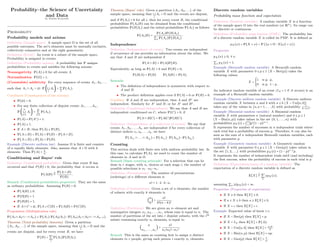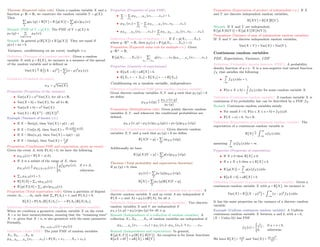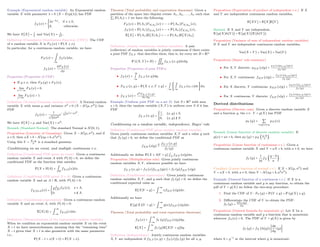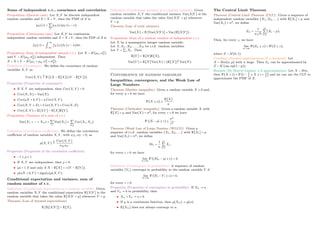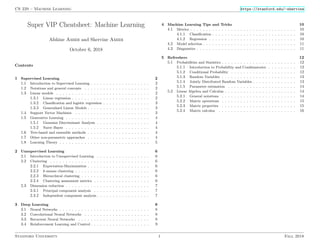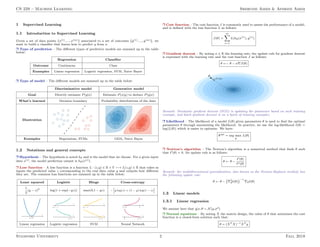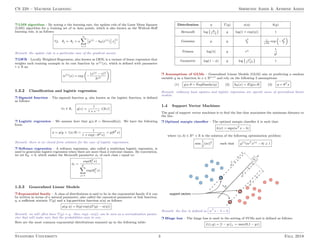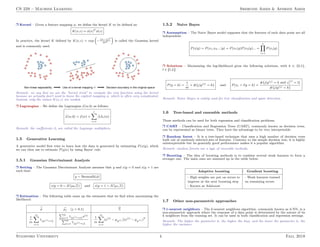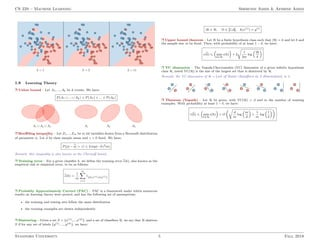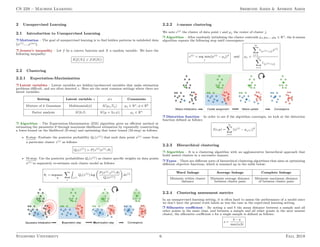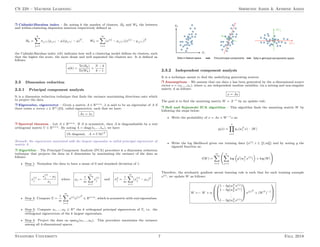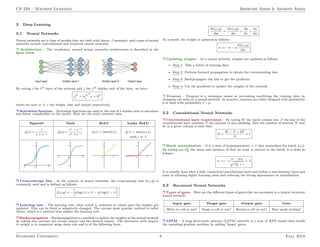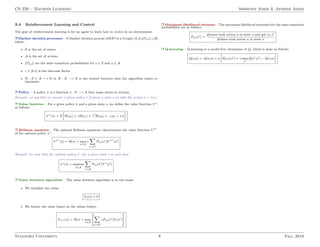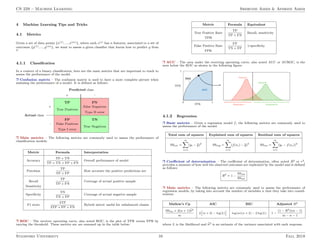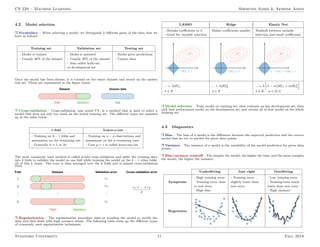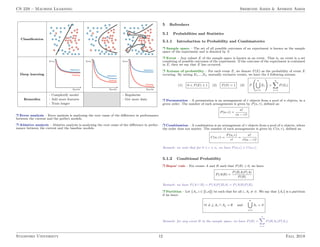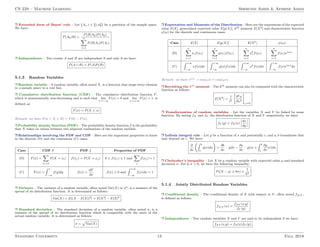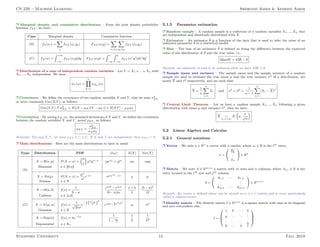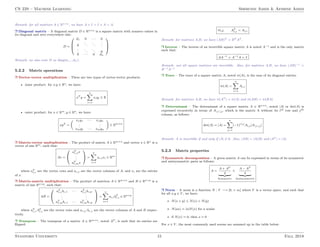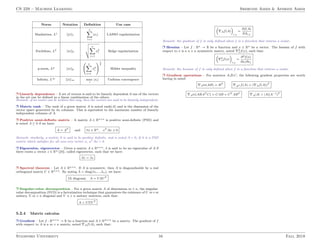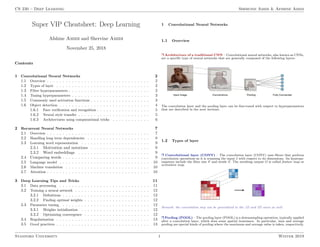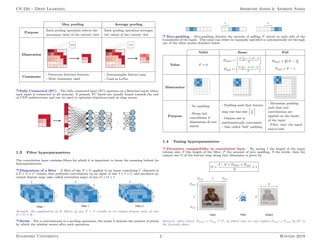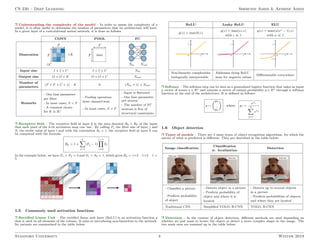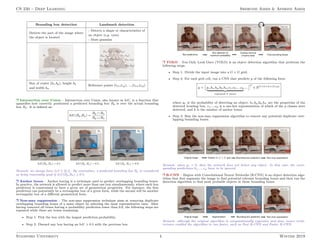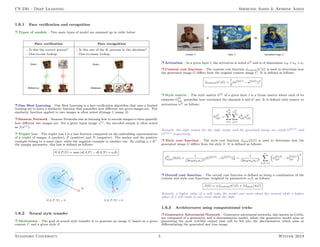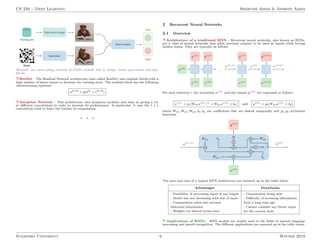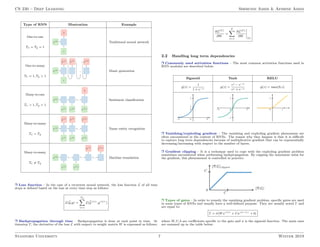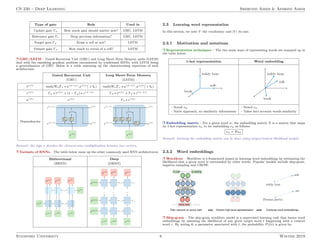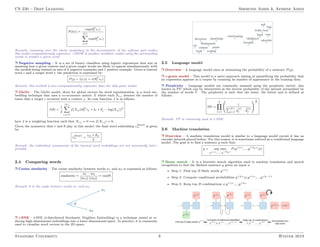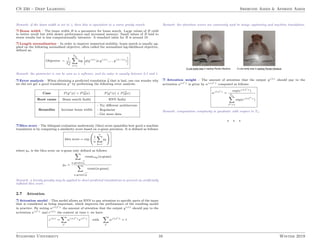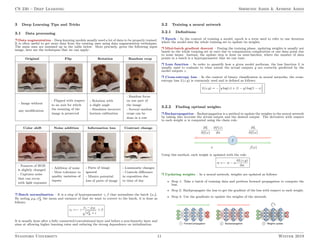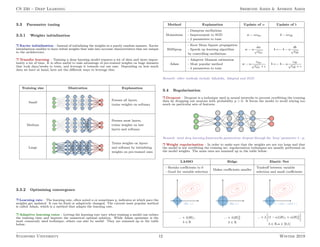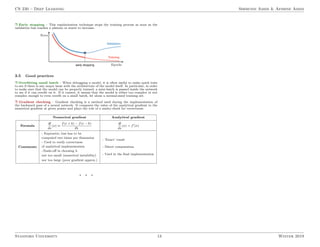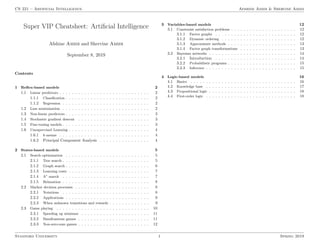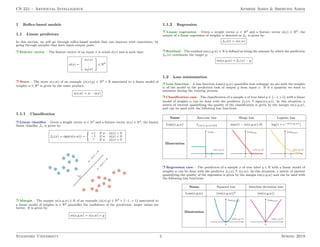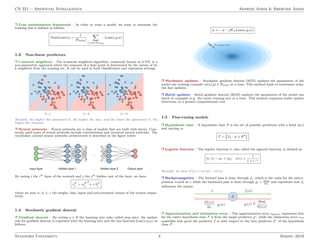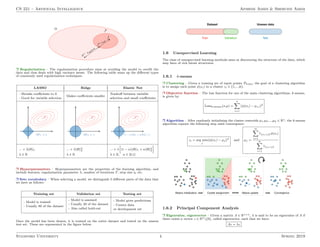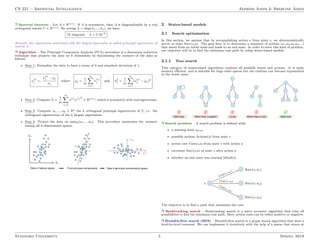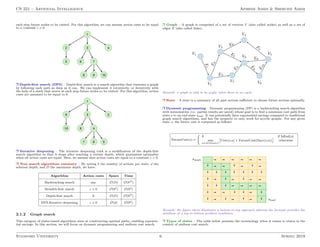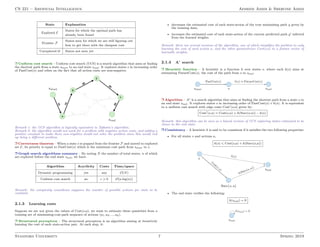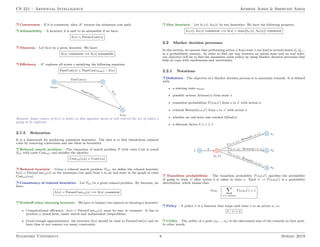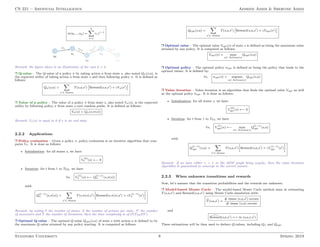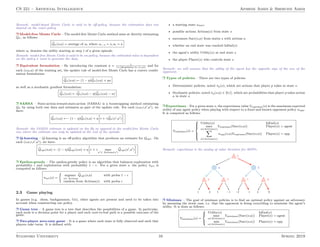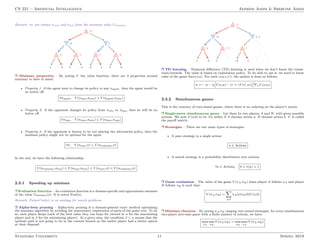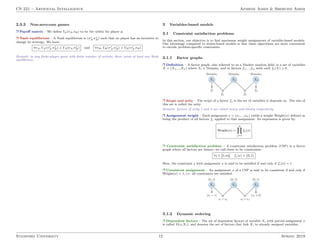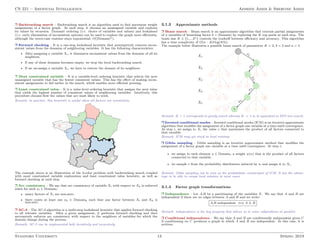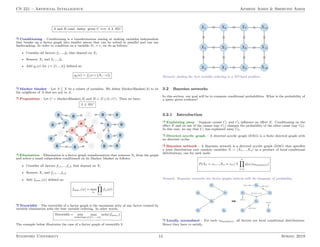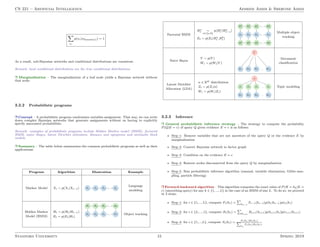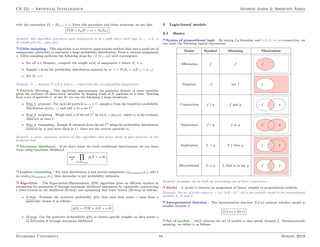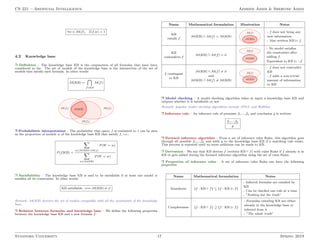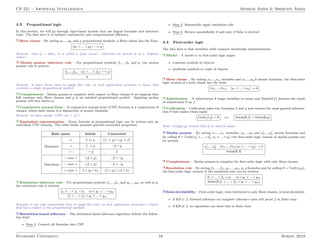1 of 52

### Deep learning .pdf

• 1. B Y S T A N F O R D U N I V E R S I T Y A N D M A S S A C H U S E T T S I N S T I T U T E O F T E C H N O L O G Y All Cheat Sheets Machine Learning, Deep Learning, Artificial Intelligence COMPILED BY - NIKHIL YADAV
• 2. Probability–the Science of Uncertainty and Data by Fabián Kozynski Probability Probability models and axioms Definition (Sample space) A sample space Ω is the set of all possible outcomes. The set’s elements must be mutually exclusive, collectively exhaustive and at the right granularity. Definition (Event) An event is a subset of the sample space. Probability is assigned to events. Definition (Probability axioms) A probability law P assigns probabilities to events and satisfies the following axioms: Nonnegativity P(A) ≥ 0 for all events A. Normalization P(Ω) = 1. (Countable) additivity For every sequence of events A1, A2, . . . such that Ai ∩ Aj = ∅: P (⋃ i Ai) = ∑ i P(Ai). Corollaries (Consequences of the axioms) • P(∅) = 0. • For any finite collection of disjoint events A1, . . . , An, P ( n ⋃ i=1 Ai) = n ∑ i=1 P(Ai). • P(A) + P(Ac) = 1. • P(A) ≤ 1. • If A ⊂ B, then P(A) ≤ P(B). • P(A ∪ B) = P(A) + P(B) − P(A ∩ B). • P(A ∪ B) ≤ P(A) + P(B). Example (Discrete uniform law) Assume Ω is finite and consists of n equally likely elements. Also, assume that A ⊂ Ω with k elements. Then P(A) = k n . Conditioning and Bayes’ rule Definition (Conditional probability) Given that event B has occurred and that P(B) > 0, the probability that A occurs is P(A∣B) △ = P(A ∩ B) P(B) . Remark (Conditional probabilities properties) They are the same as ordinary probabilities. Assuming P(B) > 0: • P(A∣B) ≥ 0. • P(Ω∣B) = 1 • P(B∣B) = 1. • If A ∩ C = ∅, P(A ∪ C∣B) = P(A∣B) + P(C∣B). Proposition (Multiplication rule) P(A1 ∩A2 ∩⋯∩An) = P(A1)⋅P(A2∣A1)⋯P(An∣A1 ∩A2 ∩⋯∩An−1). Theorem (Total probability theorem) Given a partition {A1, A2, . . .} of the sample space, meaning that ⋃ i Ai = Ω and the events are disjoint, and for every event B, we have P(B) = ∑ i P(Ai)P(B∣Ai). Theorem (Bayes’ rule) Given a partition {A1, A2, . . .} of the sample space, meaning that ⋃ i Ai = Ω and the events are disjoint, and if P(Ai) > 0 for all i, then for every event B, the conditional probabilities P(Ai∣B) can be obtained from the conditional probabilities P(B∣Ai) and the initial probabilities P(Ai) as follows: P(Ai∣B) = P(Ai)P(B∣Ai) ∑j P(Aj)P(B∣Aj) . Independence Definition (Independence of events) Two events are independent if occurrence of one provides no information about the other. We say that A and B are independent if P(A ∩ B) = P(A)P(B). Equivalently, as long as P(A) > 0 and P(B) > 0, P(B∣A) = P(B) P(A∣B) = P(A). Remarks • The definition of independence is symmetric with respect to A and B. • The product definition applies even if P(A) = 0 or P(B) = 0. Corollary If A and B are independent, then A and Bc are independent. Similarly for Ac and B, or for Ac and Bc. Definition (Conditional independence) We say that A and B are independent conditioned on C, where P(C) > 0, if P(A ∩ B∣C) = P(A∣C)P(B∣C). Definition (Independence of a collection of events) We say that events A1, A2, . . . , An are independent if for every collection of distinct indices i1, i2, . . . , ik, we have P(Ai1 ∩ . . . ∩ Aik ) = P(Ai1 ) ⋅ P(Ai2 )⋯P(Aik ). Counting This section deals with finite sets with uniform probability law. In this case, to calculate P(A), we need to count the number of elements in A and in Ω. Remark (Basic counting principle) For a selection that can be done in r stages, with ni choices at each stage i, the number of possible selections is n1 ⋅ n2⋯nr. Definition (Permutations) The number of permutations (orderings) of n different elements is n! = 1 ⋅ 2 ⋅ 3⋯n. Definition (Combinations) Given a set of n elements, the number of subsets with exactly k elements is ( n k ) = n! k!(n − k)! . Definition (Partitions) We are given an n−element set and nonnegative integers n1, n2, . . . , nr, whose sum is equal to n. The number of partitions of the set into r disjoint subsets, with the ith subset containing exactly ni elements, is equal to ( n n1, . . . , nr ) = n! n1!n2!⋯nr! . Remark This is the same as counting how to assign n distinct elements to r people, giving each person i exactly ni elements. Discrete random variables Probability mass function and expectation Definition (Random variable) A random variable X is a function of the sample space Ω into the real numbers (or Rn). Its range can be discrete or continuous. Definition (Probability mass funtion (PMF)) The probability law of a discrete random variable X is called its PMF. It is defined as pX (x) = P(X = x) = P ({ω ∈ Ω ∶ X(ω) = x}) . Properties pX (x) ≥ 0, ∀ x. ∑x pX (x) = 1. Example (Bernoulli random variable) A Bernoulli random variable X with parameter 0 ≤ p ≤ 1 (X ∼ Ber(p)) takes the following values: X = ⎧ ⎪ ⎪ ⎨ ⎪ ⎪ ⎩ 1 w.p. p, 0 w.p. 1 − p. An indicator random variable of an event (IA = 1 if A occurs) is an example of a Bernoulli random variable. Example (Discrete uniform random variable) A Discrete uniform random variable X between a and b with a ≤ b (X ∼ Uni[a, b]) takes any of the values in {a, a + 1, . . . , b} with probability 1 b−a+1 . Example (Binomial random variable) A Binomial random variable X with parameters n (natural number) and 0 ≤ p ≤ 1 (X ∼ Bin(n, p)) takes values in the set {0, 1, . . . , n} with probabilities pX (i) = (n i )pi(1 − p)n−i. It represents the number of successes in n independent trials where each trial has a probability of success p. Therefore, it can also be seen as the sum of n independent Bernoulli random variables, each with parameter p. Example (Geometric random variable) A Geometric random variable X with parameter 0 ≤ p ≤ 1 (X ∼ Geo(p)) takes values in the set {1, 2, . . .} with probabilities pX (i) = (1 − p)i−1p. It represents the number of independent trials until (and including) the first success, when the probability of success in each trial is p. Definition (Expectation/mean of a random variable) The expectation of a discrete random variable is defined as E[X] △ = ∑ x xpX (x). assuming ∑x ∣x∣pX (x) < ∞. Properties (Properties of expectation) • If X ≥ 0 then E[X] ≥ 0. • If a ≤ X ≤ b then a ≤ E[X] ≤ b. • If X = c then E[X] = c. Example Expected value of know r.v. • If X ∼ Ber(p) then E[X] = p. • If X = IA then E[X] = P(A). • If X ∼ Uni[a, b] then E[X] = a+b 2 . • If X ∼ Bin(n, p) then E[X] = np. • If X ∼ Geo(p) then E[X] = 1 p .
• 3. Theorem (Expected value rule) Given a random variable X and a function g ∶ R → R, we construct the random variable Y = g(X). Then ∑ y ypY (y) = E[Y ] = E [g(X)] = ∑ x g(x)pX (x). Remark (PMF of Y = g(X)) The PMF of Y = g(X) is pY (y) = ∑ x∶g(x)=y pX (x). Remark In general g (E[X]) ≠ E [g(X)]. They are equal if g(x) = ax + b. Variance, conditioning on an event, multiple r.v. Definition (Variance of a random variable) Given a random variable X with µ = E[X], its variance is a measure of the spread of the random variable and is defined as Var(X) △ = E [(X − µ)2 ] = ∑ x (x − µ)2 pX (x). Definition (Standard deviation) σX = √ Var(X). Properties (Properties of the variance) • Var(aX) = a2 Var(X), for all a ∈ R. • Var(X + b) = Var(X), for all b ∈ R. • Var(aX + b) = a2 Var(X). • Var(X) = E[X2] − (E[X])2 . Example (Variance of known r.v.) • If X ∼ Ber(p), then Var(X) = p(1 − p). • If X ∼ Uni[a, b], then Var(X) = (b−a)(b−a+2) 12 . • If X ∼ Bin(n, p), then Var(X) = np(1 − p). • If X ∼ Geo(p), then Var(X) = 1−p p2 Proposition (Conditional PMF and expectation, given an event) Given the event A, with P(A) > 0, we have the following • pX∣A(x) = P(X = x∣A). • If A is a subset of the range of X, then: pX∣A(x) △ = pX∣{X∈A}(x) = ⎧ ⎪ ⎪ ⎨ ⎪ ⎪ ⎩ 1 P(A) pX (x), if x ∈ A, 0, otherwise. • ∑x pX∣A(x) = 1. • E[X∣A] = ∑x xpX∣A(x). • E [g(X)∣A] = ∑x g(x)pX∣A(x). Proposition (Total expectation rule) Given a partition of disjoint events A1, . . . , An such that ∑i P(Ai) = 1, and P(Ai) > 0, E[X] = P(A1)E[X∣A1] + ⋯ + P(An)E[X∣An]. Definition (Memorylessness of the geometric random variable) When we condition a geometric random variable X on the event X > n we have memorylessness, meaning that the “remaining time” X − n, given that X > n, is also geometric with the same parameter. Formally, pX−n∣X>n(i) = pX (i). Definition (Joint PMF) The joint PMF of random variables X1, X2, . . . , Xn is pX1,X2,...,Xn (x1, . . . , xn) = P(X1 = x1, . . . , Xn = xn). Properties (Properties of joint PMF) • ∑ x1 ⋯ ∑ xn pX1,...,Xn (x1, . . . , xn) = 1. • pX1 (x1) = ∑ x2 ⋯ ∑ xn pX1,...,Xn (x1, x2, . . . , xn). • pX2,...,Xn (x2, . . . , xn) = ∑ x1 pX1,X2,...,Xn (x1, x2, . . . , xn). Definition (Functions of multiple r.v.) If Z = g(X1, . . . , Xn), where g ∶ Rn → R, then pZ (z) = P (g(X1, . . . , Xn) = z). Proposition (Expected value rule for multiple r.v.) Given g ∶ Rn → R, E [g(X1, . . . , Xn)] = ∑ x1,...,xn g(x1, . . . , xn)pX1,...,Xn (x1, . . . , xn). Properties (Linearity of expectations) • E[aX + b] = aE[X] + b. • E[X1 + ⋯ + Xn] = E[X1] + ⋯ + E[Xn]. Conditioning on a random variable, independence Definition (Conditional PMF given another random variable) Given discrete random variables X, Y and y such that pY (y) > 0 we define pX∣Y (x∣y) △ = pX,Y (x, y) pY (y) . Proposition (Multiplication rule) Given jointly discrete random variables X, Y , and whenever the conditional probabilities are defined, pX,Y (x, y) = pX (x)pY ∣X (y∣x) = pY (y)pX∣Y (x∣y). Definition (Conditional expectation) Given discrete random variables X, Y and y such that pY (y) > 0 we define E[X∣Y = y] = ∑ x xpX∣Y (x∣y). Additionally we have E [g(X)∣Y = y] = ∑ x g(x)pX∣Y (x∣y). Theorem (Total probability and expectation theorems) If pY (y) > 0, then pX (x) = ∑ y pY (y)pX∣Y (x∣y), E[X] = ∑ y pY (y)E[X∣Y = y]. Definition (Independence of a random variable and an event) A discrete random variable X and an event A are independent if P(X = x and A) = pX (x)P(A), for all x. Definition (Independence of two random variables) Two discrete random variables X and Y are independent if pX,Y (x, y) = pX (x)pY (y) for all x, y. Remark (Independence of a collection of random variables) A collection X1, X2, . . . , Xn of random variables are independent if pX1,...,Xn (x1, . . . , xn) = pX1 (x1)⋯pXn (xn), ∀ x1, . . . , xn. Remark (Independence and expectation) In general, E [g(X, Y )] ≠ g (E[X], E[Y ]). An exception is for linear functions: E[aX + bY ] = aE[X] + bE[Y ]. Proposition (Expectation of product of independent r.v.) If X and Y are discrete independent random variables, E[XY ] = E[X]E[Y ]. Remark If X and Y are independent, E [g(X)h(Y )] = E [g(X)] E [h(Y )]. Proposition (Variance of sum of independent random variables) IF X and Y are discrete independent random variables, Var(X + Y ) = Var(X) + Var(Y ). Continuous random variables PDF, Expectation, Variance, CDF Definition (Probability density function (PDF)) A probability density function of a r.v. X is a non-negative real valued function fX that satisfies the following • ∞ ∫ −∞ fX (x)dx = 1. • P(a ≤ X ≤ b) = b ∫ a fX (x)dx for some random variable X. Definition (Continuous random variable) A random variable X is continuous if its probability law can be described by a PDF fX . Remark Continuous random variables satisfy: • For small δ > 0, P(a ≤ X ≤ a + δ) ≈ fX (a)δ. • P(X = a) = 0, ∀a ∈ R. Definition (Expectation of a continuous random variable) The expectation of a continuous random variable is E[X] △ = ∫ ∞ −∞ xfX (x)dx. assuming ∞ ∫ −∞ ∣x∣fX (x)dx < ∞. Properties (Properties of expectation) • If X ≥ 0 then E[X] ≥ 0. • If a ≤ X ≤ b then a ≤ E[X] ≤ b. • E [g(X)] = ∞ ∫ −∞ g(x)fX (x)dx. • E[aX + b] = aE[X] + b. Definition (Variance of a continuous random variable) Given a continuous random variable X with µ = E[X], its variance is Var(X) = E [(X − µ)2 ] = ∫ ∞ −∞ (x − µ)2 fX (x)dx. It has the same properties as the variance of a discrete random variable. Example (Uniform continuous random variable) A Uniform continuous random variable X between a and b, with a < b, (X ∼ Uni(a, b)) has PDF fX (x) = ⎧ ⎪ ⎪ ⎨ ⎪ ⎪ ⎩ 1 b−a , if a < x < b, 0, otherwise. We have E[X] = a+b 2 and Var(X) = (b−a)2 12 .
• 4. Example (Exponential random variable) An Exponential random variable X with parameter λ > 0 (X ∼ Exp(λ)) has PDF fX (x) = ⎧ ⎪ ⎪ ⎨ ⎪ ⎪ ⎩ λe−λx, if x ≥ 0, 0, otherwise. We have E[X] = 1 λ and Var(X) = 1 λ2 . Definition (Cumulative Distribution Function (CDF)) The CDF of a random variable X is FX (x) = P(X ≤ x). In particular, for a continuous random variable, we have FX (x) = x ∫ −∞ fX (x)dx, fX (x) = dFX (x) dx . Properties (Properties of CDF) • If y ≥ x, then FX (y) ≥ FX (x). • lim x→−∞ FX (x) = 0. • lim x→∞ FX (x) = 1. Definition (Normal/Gaussian random variable) A Normal random variable X with mean µ and variance σ2 > 0 (X ∼ N(µ, σ2)) has PDF fX (x) = 1 √ 2πσ2 e − 1 2σ2 (x−µ)2 . We have E[X] = µ and Var(X) = σ2. Remark (Standard Normal) The standard Normal is N(0, 1). Proposition (Linearity of Gaussians) Given X ∼ N(µ, σ2), and if a ≠ 0, then aX + b ∼ N(aµ + b, a2σ2). Using this Y = X−µ σ is a standard gaussian. Conditioning on an event, and multiple continuous r.v. Definition (Conditional PDF given an event) Given a continuous random variable X and event A with P(A) > 0, we define the conditional PDF as the function that satisfies P(X ∈ B∣A) = ∫ B fX∣A(x)dx. Definition (Conditional PDF given X ∈ A) Given a continuous random variable X and an A ⊂ R, with P(A) > 0: fX∣X∈A(x) = ⎧ ⎪ ⎪ ⎨ ⎪ ⎪ ⎩ 1 P(A) fX (x), x ∈ A, 0, x / ∈ A. Definition (Conditional expectation) Given a continuous random variable X and an event A, with P(A) > 0: E[X∣A] = ∫ ∞ −∞ fX∣A(x)dx. Definition (Memorylessness of the exponential random variable) When we condition an exponential random variable X on the event X > t we have memorylessness, meaning that the “remaining time” X − t given that X > t is also geometric with the same parameter i.e., P(X − t > x∣X > t) = P(X > x). Theorem (Total probability and expectation theorems) Given a partition of the space into disjoint events A1, A2, . . . , An such that ∑i P(Ai) = 1 we have the following: FX (x) = P(A1)FX∣A1 (x) + ⋯ + P(An)FX∣An (x), fX (x) = P(A1)fX∣A1 (x) + ⋯ + P(An)fX∣An (x), E[X] = P(A1)E[X∣A1] + ⋯ + P(An)E[X∣An]. Definition (Jointly continuous random variables) A pair (collection) of random variables is jointly continuous if there exists a joint PDF fX,Y that describes them, that is, for every set B ⊂ Rn P ((X, Y ) ∈ B) = ∬ B fX,Y (x, y)dxdy. Properties (Properties of joint PDFs) • fX (x) = ∞ ∫ −∞ fX,Y (x, y)dy. • FX,Y (x, y) = P(X ≤ x, Y ≤ y) = x ∫ −∞ [ y ∫ −∞ fX,Y (u, v)dv] du. • fX,Y (x) = ∂2 FX,Y (x,y) ∂x ∂y . Example (Uniform joint PDF on a set S) Let S ⊂ R2 with area s > 0, then the random variable (X, Y ) is uniform over S if it has PDF fX,Y (x, y) = ⎧ ⎪ ⎪ ⎨ ⎪ ⎪ ⎩ 1 s , (x, y) ∈ S, 0, (x, y) / ∈ S. Conditioning on a random variable, independence, Bayes’ rule Definition (Conditional PDF given another random variable) Given jointly continuous random variables X, Y and a value y such that fY (y) > 0, we define the conditional PDF as fX∣Y (x∣y) △ = fX,Y (x, y) fY (y) . Additionally we define P(X ∈ A∣Y = y) ∫A fX∣Y (x∣y)dx. Proposition (Multiplication rule) Given jointly continuous random variables X, Y , whenever possible we have fX,Y (x, y) = fX (x)fY ∣X (y∣x) = fY (y)fX∣Y (x∣y). Definition (Conditional expectation) Given jointly continuous random variables X, Y , and y such that fY (y) > 0, we define the conditional expected value as E[X∣Y = y] = ∫ ∞ −∞ xfX∣Y (x∣y)dx. Additionally we have E [g(X)∣Y = y] = ∫ ∞ −∞ g(x)fX∣Y (x∣y)dx. Theorem (Total probability and total expectation theorems) fX (x) = ∫ ∞ −∞ fY (y)fX∣Y (x∣y)dy, E[X] = ∫ ∞ −∞ fY (y)E[X∣Y = y]dy. Definition (Independence) Jointly continuous random variables X, Y are independent if fX,Y (x, y) = fX (x)fY (y) for all x, y. Proposition (Expectation of product of independent r.v.) If X and Y are independent continuous random variables, E[XY ] = E[X]E[Y ]. Remark If X and Y are independent, E [g(X)h(Y )] = E [g(X)] E [h(Y )]. Proposition (Variance of sum of independent random variables) If X and Y are independent continuous random variables, Var(X + Y ) = Var(X) + Var(Y ). Proposition (Bayes’ rule summary) • For X, Y discrete: pX∣Y (x∣y) = pX (x)pY ∣X (y∣x) pY (y) . • For X, Y continuous: fX∣Y (x∣y) = fX (x)fY ∣X (y∣x) fY (y) . • For X discrete, Y continuous: pX∣Y (x∣y) = pX (x)fY ∣X (y∣x) fY (y) . • For X continuous, Y discrete: fX∣Y (x∣y) = fX (x)pY ∣X (y∣x) pY (y) . Derived distributions Proposition (Discrete case) Given a discrete random variable X and a function g, the r.v. Y = g(X) has PMF pY (y) = ∑ x∶g(x)=y pX (x). Remark (Linear function of discrete random variable) If g(x) = ax + b, then pY (y) = pX (y−b a ). Proposition (Linear function of continuous r.v.) Given a continuous random variable X and Y = aX + b, with a ≠ 0, we have fY (y) = 1 ∣a∣ fX ( y − b a ) . Corollary (Linear function of normal r.v.) If X ∼ N(µ, σ2) and Y = aX + b, with a ≠ 0, then Y ∼ N(aµ + b, a2σ2). Example (General function of a continuous r.v.) If X is a continuous random variable and g is any function, to obtain the pdf of Y = g(X) we follow the two-step procedure: 1. Find the CDF of Y : FY (y) = P(Y ≤ y) = P (g(X) ≤ y). 2. Differentiate the CDF of Y to obtain the PDF: fY (y) = dFY (y) dy . Proposition (General formula for monotonic g) Let X be a continuous random variable and g a function that is monotonic wherever fX (x) > 0. The PDF of Y = g(X) is given by fY (y) = fX (h(y)) ∣ dh dy (y)∣ . where h = g−1 in the interval where g is monotonic.
• 5. Sums of independent r.v., covariance and correlation Proposition (Discrete case) Let X, Y be discrete independent random variables and Z = X + Y , then the PMF of Z is pZ (z) = ∑ x pX (x)pY (z − x). Proposition (Continuous case) Let X, Y be continuous independent random variables and Z = X +Y , then the PDF of Z is fZ (z) = ∫ ∞ −∞ fX (x)fY (z − x)dx. Proposition (Sum of independent normal r.v.) Let X ∼ N(µx, σ2 x) and Y ∼ N(µy, σ2 y) independent. Then Z = X + Y ∼ N(µx + µy, σ2 x + σ2 y). Definition (Covariance) We define the covariance of random variables X, Y as Cov(X, Y ) △ = E [(X − E[X]) (Y − E[Y ])] . Properties (Properties of covariance) • If X, Y are independent, then Cov(X, Y ) = 0. • Cov(X, X) = Var(X). • Cov(aX + b, Y ) = a Cov(X, Y ). • Cov(X, Y + Z) = Cov(X, Y ) + Cov(X, Z). • Cov(X, Y ) = E[XY ] − E[X]E[Y ]. Proposition (Variance of a sum of r.v.) Var(X1 + ⋯ + Xn) = ∑ i Var(Xi) + ∑ i≠j Cov(Xi, Xj). Definition (Correlation coefficient) We define the correlation coefficient of random variables X, Y , with σX , σY > 0, as ρ(X, Y ) △ = Cov(X, Y ) σX σY . Properties (Properties of the correlation coefficient) • −1 ≤ ρ ≤ 1. • If X, Y are independent, then ρ = 0. • ∣ρ∣ = 1 if and only if X − E[X] = c (Y − E[Y ]). • ρ(aX + b, Y ) = sign(a)ρ(X, Y ). Conditional expectation and variance, sum of random number of r.v. Definition (Conditional expectation as a random variable) Given random variables X, Y the conditional expectation E[X∣Y ] is the random variable that takes the value E[X∣Y = y] whenever Y = y. Theorem (Law of iterated expectations) E [E[X∣Y ]] = E[X]. Definition (Conditional variance as a random variable) Given random variables X, Y the conditional variance Var(X∣Y ) is the random variable that takes the value Var(X∣Y = y) whenever Y = y. Theorem (Law of total variance) Var(X) = E [Var(X∣Y )] + Var (E[X∣Y ]) . Proposition (Sum of a random number of independent r.v.) Let N be a nonnegative integer random variable. Let X, X1, X2, . . . , XN be i.i.d. random variables. Let Y = ∑i Xi. Then E[Y ] = E[N]E[X], Var(Y ) = E[N] Var(X) + (E[X])2 Var(N). Convergence of random variables Inequalities, convergence, and the Weak Law of Large Numbers Theorem (Markov inequality) Given a random variable X ≥ 0 and, for every a > 0 we have P(X ≥ a) ≤ E[X] a . Theorem (Chebyshev inequality) Given a random variable X with E[X] = µ and Var(X) = σ2, for every 0 we have P (∣X − µ∣ ≥ ) ≤ σ2 2 . Theorem (Weak Law of Large Number (WLLN)) Given a sequence of i.i.d. random variables {X1, X2, . . .} with E[Xi] = µ and Var(Xi) = σ2, we define Mn = 1 n n ∑ i=1 Xi, for every 0 we have lim n→∞ P (∣Mn − µ∣ ≥ ) = 0. Definition (Convergence in probability) A sequence of random variables {Yi} converges in probability to the random variable Y if lim n→∞ P (∣Yi − Y ∣ ≥ ) = 0, for every 0. Properties (Properties of convergence in probability) If Xn → a and Yn → b in probability, then • Xn + Yn → a + b. • If g is a continuous function, then g(Xn) → g(a). • E[Xn] does not always converge to a. The Central Limit Theorem Theorem (Central Limit Theorem (CLT)) Given a sequence of independent random variables {X1, X2, . . .} with E[Xi] = µ and Var(Xi) = σ2, we define Zn = 1 σ √ n n ∑ i=1 (Xi − µ). Then, for every z, we have lim n→∞ P(Zn ≤ z) = P(Z ≤ z), where Z ∼ N(0, 1). Corollary (Normal approximation of a binomial) Let X ∼ Bin(n, p) with n large. Then Sn can be approximated by Z ∼ N (np, np(1 − p)). Remark (De Moivre-Laplace 1/2 approximation) Let X ∼ Bin, then P(X = i) = P (i − 1 2 ≤ X ≤ i + 1 2 ) and we can use the CLT to approximate the PMF of X.
• 6. CS 229 – Machine Learning https://stanford.edu/~shervine Super VIP Cheatsheet: Machine Learning Afshine Amidi and Shervine Amidi October 6, 2018 Contents 1 Supervised Learning 2 1.1 Introduction to Supervised Learning . . . . . . . . . . . . . . . . . . . 2 1.2 Notations and general concepts . . . . . . . . . . . . . . . . . . . . . 2 1.3 Linear models . . . . . . . . . . . . . . . . . . . . . . . . . . . . . . 2 1.3.1 Linear regression . . . . . . . . . . . . . . . . . . . . . . . . . 2 1.3.2 Classification and logistic regression . . . . . . . . . . . . . . . 3 1.3.3 Generalized Linear Models . . . . . . . . . . . . . . . . . . . . 3 1.4 Support Vector Machines . . . . . . . . . . . . . . . . . . . . . . . . 3 1.5 Generative Learning . . . . . . . . . . . . . . . . . . . . . . . . . . . 4 1.5.1 Gaussian Discriminant Analysis . . . . . . . . . . . . . . . . . 4 1.5.2 Naive Bayes . . . . . . . . . . . . . . . . . . . . . . . . . . . 4 1.6 Tree-based and ensemble methods . . . . . . . . . . . . . . . . . . . . 4 1.7 Other non-parametric approaches . . . . . . . . . . . . . . . . . . . . 4 1.8 Learning Theory . . . . . . . . . . . . . . . . . . . . . . . . . . . . . 5 2 Unsupervised Learning 6 2.1 Introduction to Unsupervised Learning . . . . . . . . . . . . . . . . . 6 2.2 Clustering . . . . . . . . . . . . . . . . . . . . . . . . . . . . . . . . 6 2.2.1 Expectation-Maximization . . . . . . . . . . . . . . . . . . . . 6 2.2.2 k-means clustering . . . . . . . . . . . . . . . . . . . . . . . . 6 2.2.3 Hierarchical clustering . . . . . . . . . . . . . . . . . . . . . . 6 2.2.4 Clustering assessment metrics . . . . . . . . . . . . . . . . . . 6 2.3 Dimension reduction . . . . . . . . . . . . . . . . . . . . . . . . . . . 7 2.3.1 Principal component analysis . . . . . . . . . . . . . . . . . . 7 2.3.2 Independent component analysis . . . . . . . . . . . . . . . . . 7 3 Deep Learning 8 3.1 Neural Networks . . . . . . . . . . . . . . . . . . . . . . . . . . . . . 8 3.2 Convolutional Neural Networks . . . . . . . . . . . . . . . . . . . . . 8 3.3 Recurrent Neural Networks . . . . . . . . . . . . . . . . . . . . . . . 8 3.4 Reinforcement Learning and Control . . . . . . . . . . . . . . . . . . . 9 4 Machine Learning Tips and Tricks 10 4.1 Metrics . . . . . . . . . . . . . . . . . . . . . . . . . . . . . . . . . . 10 4.1.1 Classification . . . . . . . . . . . . . . . . . . . . . . . . . . . 10 4.1.2 Regression . . . . . . . . . . . . . . . . . . . . . . . . . . . . 10 4.2 Model selection . . . . . . . . . . . . . . . . . . . . . . . . . . . . . . 11 4.3 Diagnostics . . . . . . . . . . . . . . . . . . . . . . . . . . . . . . . . 11 5 Refreshers 12 5.1 Probabilities and Statistics . . . . . . . . . . . . . . . . . . . . . . . . 12 5.1.1 Introduction to Probability and Combinatorics . . . . . . . . . 12 5.1.2 Conditional Probability . . . . . . . . . . . . . . . . . . . . . 12 5.1.3 Random Variables . . . . . . . . . . . . . . . . . . . . . . . . 13 5.1.4 Jointly Distributed Random Variables . . . . . . . . . . . . . . 13 5.1.5 Parameter estimation . . . . . . . . . . . . . . . . . . . . . . 14 5.2 Linear Algebra and Calculus . . . . . . . . . . . . . . . . . . . . . . . 14 5.2.1 General notations . . . . . . . . . . . . . . . . . . . . . . . . 14 5.2.2 Matrix operations . . . . . . . . . . . . . . . . . . . . . . . . 15 5.2.3 Matrix properties . . . . . . . . . . . . . . . . . . . . . . . . 15 5.2.4 Matrix calculus . . . . . . . . . . . . . . . . . . . . . . . . . 16 Stanford University 1 Fall 2018
• 7. CS 229 – Machine Learning Shervine Amidi Afshine Amidi 1 Supervised Learning 1.1 Introduction to Supervised Learning Given a set of data points {x(1), ..., x(m)} associated to a set of outcomes {y(1), ..., y(m)}, we want to build a classifier that learns how to predict y from x. r Type of prediction – The different types of predictive models are summed up in the table below: Regression Classifier Outcome Continuous Class Examples Linear regression Logistic regression, SVM, Naive Bayes r Type of model – The different models are summed up in the table below: Discriminative model Generative model Goal Directly estimate P(y|x) Estimate P(x|y) to deduce P(y|x) What’s learned Decision boundary Probability distributions of the data Illustration Examples Regressions, SVMs GDA, Naive Bayes 1.2 Notations and general concepts r Hypothesis – The hypothesis is noted hθ and is the model that we choose. For a given input data x(i), the model prediction output is hθ(x(i)). r Loss function – A loss function is a function L : (z,y) ∈ R × Y 7−→ L(z,y) ∈ R that takes as inputs the predicted value z corresponding to the real data value y and outputs how different they are. The common loss functions are summed up in the table below: Least squared Logistic Hinge Cross-entropy 1 2 (y − z)2 log(1 + exp(−yz)) max(0,1 − yz) − y log(z) + (1 − y) log(1 − z) Linear regression Logistic regression SVM Neural Network r Cost function – The cost function J is commonly used to assess the performance of a model, and is defined with the loss function L as follows: J(θ) = m X i=1 L(hθ(x(i) ), y(i) ) r Gradient descent – By noting α ∈ R the learning rate, the update rule for gradient descent is expressed with the learning rate and the cost function J as follows: θ ←− θ − α∇J(θ) Remark: Stochastic gradient descent (SGD) is updating the parameter based on each training example, and batch gradient descent is on a batch of training examples. r Likelihood – The likelihood of a model L(θ) given parameters θ is used to find the optimal parameters θ through maximizing the likelihood. In practice, we use the log-likelihood (θ) = log(L(θ)) which is easier to optimize. We have: θopt = arg max θ L(θ) r Newton’s algorithm – The Newton’s algorithm is a numerical method that finds θ such that 0(θ) = 0. Its update rule is as follows: θ ← θ − 0(θ) 00(θ) Remark: the multidimensional generalization, also known as the Newton-Raphson method, has the following update rule: θ ← θ − ∇2 θ(θ) −1 ∇θ(θ) 1.3 Linear models 1.3.1 Linear regression We assume here that y|x; θ ∼ N(µ,σ2) r Normal equations – By noting X the matrix design, the value of θ that minimizes the cost function is a closed-form solution such that: θ = (XT X)−1 XT y Stanford University 2 Fall 2018
• 8. CS 229 – Machine Learning Shervine Amidi Afshine Amidi r LMS algorithm – By noting α the learning rate, the update rule of the Least Mean Squares (LMS) algorithm for a training set of m data points, which is also known as the Widrow-Hoff learning rule, is as follows: ∀j, θj ← θj + α m X i=1 y(i) − hθ(x(i) ) x (i) j Remark: the update rule is a particular case of the gradient ascent. r LWR – Locally Weighted Regression, also known as LWR, is a variant of linear regression that weights each training example in its cost function by w(i)(x), which is defined with parameter τ ∈ R as: w(i) (x) = exp − (x(i) − x)2 2τ2 1.3.2 Classification and logistic regression r Sigmoid function – The sigmoid function g, also known as the logistic function, is defined as follows: ∀z ∈ R, g(z) = 1 1 + e−z ∈]0,1[ r Logistic regression – We assume here that y|x; θ ∼ Bernoulli(φ). We have the following form: φ = p(y = 1|x; θ) = 1 1 + exp(−θT x) = g(θT x) Remark: there is no closed form solution for the case of logistic regressions. r Softmax regression – A softmax regression, also called a multiclass logistic regression, is used to generalize logistic regression when there are more than 2 outcome classes. By convention, we set θK = 0, which makes the Bernoulli parameter φi of each class i equal to: φi = exp(θT i x) K X j=1 exp(θT j x) 1.3.3 Generalized Linear Models r Exponential family – A class of distributions is said to be in the exponential family if it can be written in terms of a natural parameter, also called the canonical parameter or link function, η, a sufficient statistic T(y) and a log-partition function a(η) as follows: p(y; η) = b(y) exp(ηT(y) − a(η)) Remark: we will often have T(y) = y. Also, exp(−a(η)) can be seen as a normalization param- eter that will make sure that the probabilities sum to one. Here are the most common exponential distributions summed up in the following table: Distribution η T(y) a(η) b(y) Bernoulli log φ 1−φ y log(1 + exp(η)) 1 Gaussian µ y η2 2 1 √ 2π exp −y2 2 Poisson log(λ) y eη 1 y! Geometric log(1 − φ) y log eη 1−eη 1 r Assumptions of GLMs – Generalized Linear Models (GLM) aim at predicting a random variable y as a function fo x ∈ Rn+1 and rely on the following 3 assumptions: (1) y|x; θ ∼ ExpFamily(η) (2) hθ(x) = E[y|x; θ] (3) η = θT x Remark: ordinary least squares and logistic regression are special cases of generalized linear models. 1.4 Support Vector Machines The goal of support vector machines is to find the line that maximizes the minimum distance to the line. r Optimal margin classifier – The optimal margin classifier h is such that: h(x) = sign(wT x − b) where (w, b) ∈ Rn × R is the solution of the following optimization problem: min 1 2 ||w||2 such that y(i) (wT x(i) − b) 1 Remark: the line is defined as wT x − b = 0 . r Hinge loss – The hinge loss is used in the setting of SVMs and is defined as follows: L(z,y) = [1 − yz]+ = max(0,1 − yz) Stanford University 3 Fall 2018
• 9. CS 229 – Machine Learning Shervine Amidi Afshine Amidi r Kernel – Given a feature mapping φ, we define the kernel K to be defined as: K(x,z) = φ(x)T φ(z) In practice, the kernel K defined by K(x,z) = exp − ||x−z||2 2σ2 is called the Gaussian kernel and is commonly used. Remark: we say that we use the kernel trick to compute the cost function using the kernel because we actually don’t need to know the explicit mapping φ, which is often very complicated. Instead, only the values K(x,z) are needed. r Lagrangian – We define the Lagrangian L(w,b) as follows: L(w,b) = f(w) + l X i=1 βihi(w) Remark: the coefficients βi are called the Lagrange multipliers. 1.5 Generative Learning A generative model first tries to learn how the data is generated by estimating P(x|y), which we can then use to estimate P(y|x) by using Bayes’ rule. 1.5.1 Gaussian Discriminant Analysis r Setting – The Gaussian Discriminant Analysis assumes that y and x|y = 0 and x|y = 1 are such that: y ∼ Bernoulli(φ) x|y = 0 ∼ N(µ0,Σ) and x|y = 1 ∼ N(µ1,Σ) r Estimation – The following table sums up the estimates that we find when maximizing the likelihood: b φ b µj (j = 0,1) b Σ 1 m m X i=1 1{y(i)=1} Pm i=1 1{y(i)=j}x(i) Pm i=1 1{y(i)=j} 1 m m X i=1 (x(i) − µy(i) )(x(i) − µy(i) )T 1.5.2 Naive Bayes r Assumption – The Naive Bayes model supposes that the features of each data point are all independent: P(x|y) = P(x1,x2,...|y) = P(x1|y)P(x2|y)... = n Y i=1 P(xi|y) r Solutions – Maximizing the log-likelihood gives the following solutions, with k ∈ {0,1}, l ∈ [[1,L]] P(y = k) = 1 m × #{j|y(j) = k} and P(xi = l|y = k) = #{j|y(j) = k and x (j) i = l} #{j|y(j) = k} Remark: Naive Bayes is widely used for text classification and spam detection. 1.6 Tree-based and ensemble methods These methods can be used for both regression and classification problems. r CART – Classification and Regression Trees (CART), commonly known as decision trees, can be represented as binary trees. They have the advantage to be very interpretable. r Random forest – It is a tree-based technique that uses a high number of decision trees built out of randomly selected sets of features. Contrary to the simple decision tree, it is highly uninterpretable but its generally good performance makes it a popular algorithm. Remark: random forests are a type of ensemble methods. r Boosting – The idea of boosting methods is to combine several weak learners to form a stronger one. The main ones are summed up in the table below: Adaptive boosting Gradient boosting - High weights are put on errors to - Weak learners trained improve at the next boosting step on remaining errors - Known as Adaboost 1.7 Other non-parametric approaches r k-nearest neighbors – The k-nearest neighbors algorithm, commonly known as k-NN, is a non-parametric approach where the response of a data point is determined by the nature of its k neighbors from the training set. It can be used in both classification and regression settings. Remark: The higher the parameter k, the higher the bias, and the lower the parameter k, the higher the variance. Stanford University 4 Fall 2018
• 10. CS 229 – Machine Learning Shervine Amidi Afshine Amidi 1.8 Learning Theory r Union bound – Let A1, ..., Ak be k events. We have: P(A1 ∪ ... ∪ Ak) 6 P(A1) + ... + P(Ak) r Hoeffding inequality – Let Z1, .., Zm be m iid variables drawn from a Bernoulli distribution of parameter φ. Let b φ be their sample mean and γ 0 fixed. We have: P(|φ − b φ| γ) 6 2 exp(−2γ2 m) Remark: this inequality is also known as the Chernoff bound. r Training error – For a given classifier h, we define the training error b (h), also known as the empirical risk or empirical error, to be as follows: b (h) = 1 m m X i=1 1{h(x(i))6=y(i)} r Probably Approximately Correct (PAC) – PAC is a framework under which numerous results on learning theory were proved, and has the following set of assumptions: • the training and testing sets follow the same distribution • the training examples are drawn independently r Shattering – Given a set S = {x(1),...,x(d)}, and a set of classifiers H, we say that H shatters S if for any set of labels {y(1), ..., y(d)}, we have: ∃h ∈ H, ∀i ∈ [[1,d]], h(x(i) ) = y(i) r Upper bound theorem – Let H be a finite hypothesis class such that |H| = k and let δ and the sample size m be fixed. Then, with probability of at least 1 − δ, we have: (b h) 6 min h∈H (h) + 2 r 1 2m log 2k δ r VC dimension – The Vapnik-Chervonenkis (VC) dimension of a given infinite hypothesis class H, noted VC(H) is the size of the largest set that is shattered by H. Remark: the VC dimension of H = {set of linear classifiers in 2 dimensions} is 3. r Theorem (Vapnik) – Let H be given, with VC(H) = d and m the number of training examples. With probability at least 1 − δ, we have: (b h) 6 min h∈H (h) + O r d m log m d + 1 m log 1 δ Stanford University 5 Fall 2018
• 11. CS 229 – Machine Learning Shervine Amidi Afshine Amidi 2 Unsupervised Learning 2.1 Introduction to Unsupervised Learning r Motivation – The goal of unsupervised learning is to find hidden patterns in unlabeled data {x(1),...,x(m)}. r Jensen’s inequality – Let f be a convex function and X a random variable. We have the following inequality: E[f(X)] f(E[X]) 2.2 Clustering 2.2.1 Expectation-Maximization r Latent variables – Latent variables are hidden/unobserved variables that make estimation problems difficult, and are often denoted z. Here are the most common settings where there are latent variables: Setting Latent variable z x|z Comments Mixture of k Gaussians Multinomial(φ) N(µj,Σj) µj ∈ Rn, φ ∈ Rk Factor analysis N(0,I) N(µ + Λz,ψ) µj ∈ Rn r Algorithm – The Expectation-Maximization (EM) algorithm gives an efficient method at estimating the parameter θ through maximum likelihood estimation by repeatedly constructing a lower-bound on the likelihood (E-step) and optimizing that lower bound (M-step) as follows: • E-step: Evaluate the posterior probability Qi(z(i)) that each data point x(i) came from a particular cluster z(i) as follows: Qi(z(i) ) = P(z(i) |x(i) ; θ) • M-step: Use the posterior probabilities Qi(z(i)) as cluster specific weights on data points x(i) to separately re-estimate each cluster model as follows: θi = argmax θ X i ˆ z(i) Qi(z(i) ) log P(x(i),z(i); θ) Qi(z(i)) dz(i) 2.2.2 k-means clustering We note c(i) the cluster of data point i and µj the center of cluster j. r Algorithm – After randomly initializing the cluster centroids µ1,µ2,...,µk ∈ Rn, the k-means algorithm repeats the following step until convergence: c(i) = arg min j ||x(i) − µj||2 and µj = m X i=1 1{c(i)=j}x(i) m X i=1 1{c(i)=j} r Distortion function – In order to see if the algorithm converges, we look at the distortion function defined as follows: J(c,µ) = m X i=1 ||x(i) − µc(i) ||2 2.2.3 Hierarchical clustering r Algorithm – It is a clustering algorithm with an agglomerative hierarchical approach that build nested clusters in a successive manner. r Types – There are different sorts of hierarchical clustering algorithms that aims at optimizing different objective functions, which is summed up in the table below: Ward linkage Average linkage Complete linkage Minimize within cluster Minimize average distance Minimize maximum distance distance between cluster pairs of between cluster pairs 2.2.4 Clustering assessment metrics In an unsupervised learning setting, it is often hard to assess the performance of a model since we don’t have the ground truth labels as was the case in the supervised learning setting. r Silhouette coefficient – By noting a and b the mean distance between a sample and all other points in the same class, and between a sample and all other points in the next nearest cluster, the silhouette coefficient s for a single sample is defined as follows: s = b − a max(a,b) Stanford University 6 Fall 2018
• 12. CS 229 – Machine Learning Shervine Amidi Afshine Amidi r Calinski-Harabaz index – By noting k the number of clusters, Bk and Wk the between and within-clustering dispersion matrices respectively defined as Bk = k X j=1 nc(i) (µc(i) − µ)(µc(i) − µ)T , Wk = m X i=1 (x(i) − µc(i) )(x(i) − µc(i) )T the Calinski-Harabaz index s(k) indicates how well a clustering model defines its clusters, such that the higher the score, the more dense and well separated the clusters are. It is defined as follows: s(k) = Tr(Bk) Tr(Wk) × N − k k − 1 2.3 Dimension reduction 2.3.1 Principal component analysis It is a dimension reduction technique that finds the variance maximizing directions onto which to project the data. r Eigenvalue, eigenvector – Given a matrix A ∈ Rn×n, λ is said to be an eigenvalue of A if there exists a vector z ∈ Rn{0}, called eigenvector, such that we have: Az = λz r Spectral theorem – Let A ∈ Rn×n. If A is symmetric, then A is diagonalizable by a real orthogonal matrix U ∈ Rn×n. By noting Λ = diag(λ1,...,λn), we have: ∃Λ diagonal, A = UΛUT Remark: the eigenvector associated with the largest eigenvalue is called principal eigenvector of matrix A. r Algorithm – The Principal Component Analysis (PCA) procedure is a dimension reduction technique that projects the data on k dimensions by maximizing the variance of the data as follows: • Step 1: Normalize the data to have a mean of 0 and standard deviation of 1. x (i) j ← x (i) j − µj σj where µj = 1 m m X i=1 x (i) j and σ2 j = 1 m m X i=1 (x (i) j − µj)2 • Step 2: Compute Σ = 1 m m X i=1 x(i) x(i)T ∈ Rn×n , which is symmetric with real eigenvalues. • Step 3: Compute u1, ..., uk ∈ Rn the k orthogonal principal eigenvectors of Σ, i.e. the orthogonal eigenvectors of the k largest eigenvalues. • Step 4: Project the data on spanR(u1,...,uk). This procedure maximizes the variance among all k-dimensional spaces. 2.3.2 Independent component analysis It is a technique meant to find the underlying generating sources. r Assumptions – We assume that our data x has been generated by the n-dimensional source vector s = (s1,...,sn), where si are independent random variables, via a mixing and non-singular matrix A as follows: x = As The goal is to find the unmixing matrix W = A−1 by an update rule. r Bell and Sejnowski ICA algorithm – This algorithm finds the unmixing matrix W by following the steps below: • Write the probability of x = As = W−1s as: p(x) = n Y i=1 ps(wT i x) · |W| • Write the log likelihood given our training data {x(i), i ∈ [[1,m]]} and by noting g the sigmoid function as: l(W) = m X i=1 n X j=1 log g0 (wT j x(i) ) + log |W| ! Therefore, the stochastic gradient ascent learning rule is such that for each training example x(i), we update W as follows: W ←− W + α       1 − 2g(wT 1 x(i)) 1 − 2g(wT 2 x(i)) . . . 1 − 2g(wT n x(i))   x(i)T + (WT )−1    Stanford University 7 Fall 2018
• 13. CS 229 – Machine Learning Shervine Amidi Afshine Amidi 3 Deep Learning 3.1 Neural Networks Neural networks are a class of models that are built with layers. Commonly used types of neural networks include convolutional and recurrent neural networks. r Architecture – The vocabulary around neural networks architectures is described in the figure below: By noting i the ith layer of the network and j the jth hidden unit of the layer, we have: z [i] j = w [i] j T x + b [i] j where we note w, b, z the weight, bias and output respectively. r Activation function – Activation functions are used at the end of a hidden unit to introduce non-linear complexities to the model. Here are the most common ones: Sigmoid Tanh ReLU Leaky ReLU g(z) = 1 1 + e−z g(z) = ez − e−z ez + e−z g(z) = max(0,z) g(z) = max(z,z) with 1 r Cross-entropy loss – In the context of neural networks, the cross-entropy loss L(z,y) is commonly used and is defined as follows: L(z,y) = − h y log(z) + (1 − y) log(1 − z) i r Learning rate – The learning rate, often noted η, indicates at which pace the weights get updated. This can be fixed or adaptively changed. The current most popular method is called Adam, which is a method that adapts the learning rate. r Backpropagation – Backpropagation is a method to update the weights in the neural network by taking into account the actual output and the desired output. The derivative with respect to weight w is computed using chain rule and is of the following form: ∂L(z,y) ∂w = ∂L(z,y) ∂a × ∂a ∂z × ∂z ∂w As a result, the weight is updated as follows: w ←− w − η ∂L(z,y) ∂w r Updating weights – In a neural network, weights are updated as follows: • Step 1: Take a batch of training data. • Step 2: Perform forward propagation to obtain the corresponding loss. • Step 3: Backpropagate the loss to get the gradients. • Step 4: Use the gradients to update the weights of the network. r Dropout – Dropout is a technique meant at preventing overfitting the training data by dropping out units in a neural network. In practice, neurons are either dropped with probability p or kept with probability 1 − p. 3.2 Convolutional Neural Networks r Convolutional layer requirement – By noting W the input volume size, F the size of the convolutional layer neurons, P the amount of zero padding, then the number of neurons N that fit in a given volume is such that: N = W − F + 2P S + 1 r Batch normalization – It is a step of hyperparameter γ, β that normalizes the batch {xi}. By noting µB, σ2 B the mean and variance of that we want to correct to the batch, it is done as follows: xi ←− γ xi − µB p σ2 B + + β It is usually done after a fully connected/convolutional layer and before a non-linearity layer and aims at allowing higher learning rates and reducing the strong dependence on initialization. 3.3 Recurrent Neural Networks r Types of gates – Here are the different types of gates that we encounter in a typical recurrent neural network: Input gate Forget gate Output gate Gate Write to cell or not? Erase a cell or not? Reveal a cell or not? How much writing? r LSTM – A long short-term memory (LSTM) network is a type of RNN model that avoids the vanishing gradient problem by adding ’forget’ gates. Stanford University 8 Fall 2018
• 14. CS 229 – Machine Learning Shervine Amidi Afshine Amidi 3.4 Reinforcement Learning and Control The goal of reinforcement learning is for an agent to learn how to evolve in an environment. r Markov decision processes – A Markov decision process (MDP) is a 5-tuple (S,A,{Psa},γ,R) where: • S is the set of states • A is the set of actions • {Psa} are the state transition probabilities for s ∈ S and a ∈ A • γ ∈ [0,1[ is the discount factor • R : S × A −→ R or R : S −→ R is the reward function that the algorithm wants to maximize r Policy – A policy π is a function π : S −→ A that maps states to actions. Remark: we say that we execute a given policy π if given a state s we take the action a = π(s). r Value function – For a given policy π and a given state s, we define the value function V π as follows: V π (s) = E h R(s0) + γR(s1) + γ2 R(s2) + ...|s0 = s,π i r Bellman equation – The optimal Bellman equations characterizes the value function V π∗ of the optimal policy π∗: V π∗ (s) = R(s) + max a∈A γ X s0∈S Psa(s0 )V π∗ (s0 ) Remark: we note that the optimal policy π∗ for a given state s is such that: π∗ (s) = argmax a∈A X s0∈S Psa(s0 )V ∗ (s0 ) r Value iteration algorithm – The value iteration algorithm is in two steps: • We initialize the value: V0(s) = 0 • We iterate the value based on the values before: Vi+1(s) = R(s) + max a∈A X s0∈S γPsa(s0 )Vi(s0 ) # r Maximum likelihood estimate – The maximum likelihood estimates for the state transition probabilities are as follows: Psa(s0 ) = #times took action a in state s and got to s0 #times took action a in state s r Q-learning – Q-learning is a model-free estimation of Q, which is done as follows: Q(s,a) ← Q(s,a) + α h R(s,a,s0 ) + γ max a0 Q(s0 ,a0 ) − Q(s,a) i Stanford University 9 Fall 2018
• 15. CS 229 – Machine Learning Shervine Amidi Afshine Amidi 4 Machine Learning Tips and Tricks 4.1 Metrics Given a set of data points {x(1), ..., x(m)}, where each x(i) has n features, associated to a set of outcomes {y(1), ..., y(m)}, we want to assess a given classifier that learns how to predict y from x. 4.1.1 Classification In a context of a binary classification, here are the main metrics that are important to track to assess the performance of the model. r Confusion matrix – The confusion matrix is used to have a more complete picture when assessing the performance of a model. It is defined as follows: Predicted class + – Actual class TP FN + False Negatives True Positives Type II error FP TN – False Positives True Negatives Type I error r Main metrics – The following metrics are commonly used to assess the performance of classification models: Metric Formula Interpretation Accuracy TP + TN TP + TN + FP + FN Overall performance of model Precision TP TP + FP How accurate the positive predictions are Recall TP TP + FN Coverage of actual positive sample Sensitivity Specificity TN TN + FP Coverage of actual negative sample F1 score 2TP 2TP + FP + FN Hybrid metric useful for unbalanced classes r ROC – The receiver operating curve, also noted ROC, is the plot of TPR versus FPR by varying the threshold. These metrics are are summed up in the table below: Metric Formula Equivalent True Positive Rate TP TP + FN Recall, sensitivity TPR False Positive Rate FP TN + FP 1-specificity FPR r AUC – The area under the receiving operating curve, also noted AUC or AUROC, is the area below the ROC as shown in the following figure: 4.1.2 Regression r Basic metrics – Given a regression model f, the following metrics are commonly used to assess the performance of the model: Total sum of squares Explained sum of squares Residual sum of squares SStot = m X i=1 (yi − y)2 SSreg = m X i=1 (f(xi) − y)2 SSres = m X i=1 (yi − f(xi))2 r Coefficient of determination – The coefficient of determination, often noted R2 or r2, provides a measure of how well the observed outcomes are replicated by the model and is defined as follows: R2 = 1 − SSres SStot r Main metrics – The following metrics are commonly used to assess the performance of regression models, by taking into account the number of variables n that they take into consid- eration: Mallow’s Cp AIC BIC Adjusted R2 SSres + 2(n + 1)b σ2 m 2 (n + 2) − log(L) log(m)(n + 2) − 2 log(L) 1 − (1 − R2)(m − 1) m − n − 1 where L is the likelihood and b σ2 is an estimate of the variance associated with each response. Stanford University 10 Fall 2018
• 16. CS 229 – Machine Learning Shervine Amidi Afshine Amidi 4.2 Model selection r Vocabulary – When selecting a model, we distinguish 3 different parts of the data that we have as follows: Training set Validation set Testing set - Model is trained - Model is assessed - Model gives predictions - Usually 80% of the dataset - Usually 20% of the dataset - Unseen data - Also called hold-out or development set Once the model has been chosen, it is trained on the entire dataset and tested on the unseen test set. These are represented in the figure below: r Cross-validation – Cross-validation, also noted CV, is a method that is used to select a model that does not rely too much on the initial training set. The different types are summed up in the table below: k-fold Leave-p-out - Training on k − 1 folds and - Training on n − p observations and assessment on the remaining one assessment on the p remaining ones - Generally k = 5 or 10 - Case p = 1 is called leave-one-out The most commonly used method is called k-fold cross-validation and splits the training data into k folds to validate the model on one fold while training the model on the k − 1 other folds, all of this k times. The error is then averaged over the k folds and is named cross-validation error. r Regularization – The regularization procedure aims at avoiding the model to overfit the data and thus deals with high variance issues. The following table sums up the different types of commonly used regularization techniques: LASSO Ridge Elastic Net - Shrinks coefficients to 0 Makes coefficients smaller Tradeoff between variable - Good for variable selection selection and small coefficients ... + λ||θ||1 ... + λ||θ||2 2 ... + λ h (1 − α)||θ||1 + α||θ||2 2 i λ ∈ R λ ∈ R λ ∈ R, α ∈ [0,1] r Model selection – Train model on training set, then evaluate on the development set, then pick best performance model on the development set, and retrain all of that model on the whole training set. 4.3 Diagnostics r Bias – The bias of a model is the difference between the expected prediction and the correct model that we try to predict for given data points. r Variance – The variance of a model is the variability of the model prediction for given data points. r Bias/variance tradeoff – The simpler the model, the higher the bias, and the more complex the model, the higher the variance. Underfitting Just right Overfitting - High training error - Training error - Low training error Symptoms - Training error close slightly lower than - Training error much to test error test error lower than test error - High bias - High variance Regression Stanford University 11 Fall 2018
• 17. CS 229 – Machine Learning Shervine Amidi Afshine Amidi Classification Deep learning - Complexify model - Regularize Remedies - Add more features - Get more data - Train longer r Error analysis – Error analysis is analyzing the root cause of the difference in performance between the current and the perfect models. r Ablative analysis – Ablative analysis is analyzing the root cause of the difference in perfor- mance between the current and the baseline models. 5 Refreshers 5.1 Probabilities and Statistics 5.1.1 Introduction to Probability and Combinatorics r Sample space – The set of all possible outcomes of an experiment is known as the sample space of the experiment and is denoted by S. r Event – Any subset E of the sample space is known as an event. That is, an event is a set consisting of possible outcomes of the experiment. If the outcome of the experiment is contained in E, then we say that E has occurred. r Axioms of probability – For each event E, we denote P(E) as the probability of event E occuring. By noting E1,...,En mutually exclusive events, we have the 3 following axioms: (1) 0 6 P(E) 6 1 (2) P(S) = 1 (3) P n [ i=1 Ei ! = n X i=1 P(Ei) r Permutation – A permutation is an arrangement of r objects from a pool of n objects, in a given order. The number of such arrangements is given by P(n, r), defined as: P(n, r) = n! (n − r)! r Combination – A combination is an arrangement of r objects from a pool of n objects, where the order does not matter. The number of such arrangements is given by C(n, r), defined as: C(n, r) = P(n, r) r! = n! r!(n − r)! Remark: we note that for 0 6 r 6 n, we have P(n,r) C(n,r). 5.1.2 Conditional Probability r Bayes’ rule – For events A and B such that P(B) 0, we have: P(A|B) = P(B|A)P(A) P(B) Remark: we have P(A ∩ B) = P(A)P(B|A) = P(A|B)P(B). r Partition – Let {Ai, i ∈ [[1,n]]} be such that for all i, Ai 6= ∅. We say that {Ai} is a partition if we have: ∀i 6= j, Ai ∩ Aj = ∅ and n [ i=1 Ai = S Remark: for any event B in the sample space, we have P(B) = n X i=1 P(B|Ai)P(Ai). Stanford University 12 Fall 2018
• 18. CS 229 – Machine Learning Shervine Amidi Afshine Amidi r Extended form of Bayes’ rule – Let {Ai, i ∈ [[1,n]]} be a partition of the sample space. We have: P(Ak|B) = P(B|Ak)P(Ak) n X i=1 P(B|Ai)P(Ai) r Independence – Two events A and B are independent if and only if we have: P(A ∩ B) = P(A)P(B) 5.1.3 Random Variables r Random variable – A random variable, often noted X, is a function that maps every element in a sample space to a real line. r Cumulative distribution function (CDF) – The cumulative distribution function F, which is monotonically non-decreasing and is such that lim x→−∞ F(x) = 0 and lim x→+∞ F(x) = 1, is defined as: F(x) = P(X 6 x) Remark: we have P(a X 6 B) = F(b) − F(a). r Probability density function (PDF) – The probability density function f is the probability that X takes on values between two adjacent realizations of the random variable. r Relationships involving the PDF and CDF – Here are the important properties to know in the discrete (D) and the continuous (C) cases. Case CDF F PDF f Properties of PDF (D) F(x) = X xi6x P(X = xi) f(xj) = P(X = xj) 0 6 f(xj) 6 1 and X j f(xj) = 1 (C) F(x) = ˆ x −∞ f(y)dy f(x) = dF dx f(x) 0 and ˆ +∞ −∞ f(x)dx = 1 r Variance – The variance of a random variable, often noted Var(X) or σ2, is a measure of the spread of its distribution function. It is determined as follows: Var(X) = E[(X − E[X])2 ] = E[X2 ] − E[X]2 r Standard deviation – The standard deviation of a random variable, often noted σ, is a measure of the spread of its distribution function which is compatible with the units of the actual random variable. It is determined as follows: σ = p Var(X) r Expectation and Moments of the Distribution – Here are the expressions of the expected value E[X], generalized expected value E[g(X)], kth moment E[Xk] and characteristic function ψ(ω) for the discrete and continuous cases: Case E[X] E[g(X)] E[Xk] ψ(ω) (D) n X i=1 xif(xi) n X i=1 g(xi)f(xi) n X i=1 xk i f(xi) n X i=1 f(xi)eiωxi (C) ˆ +∞ −∞ xf(x)dx ˆ +∞ −∞ g(x)f(x)dx ˆ +∞ −∞ xk f(x)dx ˆ +∞ −∞ f(x)eiωx dx Remark: we have eiωx = cos(ωx) + i sin(ωx). r Revisiting the kth moment – The kth moment can also be computed with the characteristic function as follows: E[Xk ] = 1 ik ∂kψ ∂ωk ω=0 r Transformation of random variables – Let the variables X and Y be linked by some function. By noting fX and fY the distribution function of X and Y respectively, we have: fY (y) = fX (x) dx dy r Leibniz integral rule – Let g be a function of x and potentially c, and a, b boundaries that may depend on c. We have: ∂ ∂c ˆ b a g(x)dx = ∂b ∂c · g(b) − ∂a ∂c · g(a) + ˆ b a ∂g ∂c (x)dx r Chebyshev’s inequality – Let X be a random variable with expected value µ and standard deviation σ. For k, σ 0, we have the following inequality: P(|X − µ| kσ) 6 1 k2 5.1.4 Jointly Distributed Random Variables r Conditional density – The conditional density of X with respect to Y , often noted fX|Y , is defined as follows: fX|Y (x) = fXY (x,y) fY (y) r Independence – Two random variables X and Y are said to be independent if we have: fXY (x,y) = fX (x)fY (y) Stanford University 13 Fall 2018
• 19. CS 229 – Machine Learning Shervine Amidi Afshine Amidi r Marginal density and cumulative distribution – From the joint density probability function fXY , we have: Case Marginal density Cumulative function (D) fX (xi) = X j fXY (xi,yj) FXY (x,y) = X xi6x X yj 6y fXY (xi,yj) (C) fX (x) = ˆ +∞ −∞ fXY (x,y)dy FXY (x,y) = ˆ x −∞ ˆ y −∞ fXY (x0 ,y0 )dx0 dy0 r Distribution of a sum of independent random variables – Let Y = X1 + ... + Xn with X1, ..., Xn independent. We have: ψY (ω) = n Y k=1 ψXk (ω) r Covariance – We define the covariance of two random variables X and Y , that we note σ2 XY or more commonly Cov(X,Y ), as follows: Cov(X,Y ) , σ2 XY = E[(X − µX )(Y − µY )] = E[XY ] − µX µY r Correlation – By noting σX , σY the standard deviations of X and Y , we define the correlation between the random variables X and Y , noted ρXY , as follows: ρXY = σ2 XY σX σY Remarks: For any X, Y , we have ρXY ∈ [−1,1]. If X and Y are independent, then ρXY = 0. r Main distributions – Here are the main distributions to have in mind: Type Distribution PDF ψ(ω) E[X] Var(X) X ∼ B(n, p) P(X = x) = n x px qn−x (peiω + q)n np npq Binomial x ∈ [[0,n]] (D) X ∼ Po(µ) P(X = x) = µx x! e−µ eµ(eiω −1) µ µ Poisson x ∈ N X ∼ U(a, b) f(x) = 1 b − a eiωb − eiωa (b − a)iω a + b 2 (b − a)2 12 Uniform x ∈ [a,b] (C) X ∼ N(µ, σ) f(x) = 1 √ 2πσ e − 1 2 x−µ σ 2 eiωµ− 1 2 ω2 σ2 µ σ2 Gaussian x ∈ R X ∼ Exp(λ) f(x) = λe−λx 1 1 − iω λ 1 λ 1 λ2 Exponential x ∈ R+ 5.1.5 Parameter estimation r Random sample – A random sample is a collection of n random variables X1, ..., Xn that are independent and identically distributed with X. r Estimator – An estimator θ̂ is a function of the data that is used to infer the value of an unknown parameter θ in a statistical model. r Bias – The bias of an estimator θ̂ is defined as being the difference between the expected value of the distribution of θ̂ and the true value, i.e.: Bias(θ̂) = E[θ̂] − θ Remark: an estimator is said to be unbiased when we have E[θ̂] = θ. r Sample mean and variance – The sample mean and the sample variance of a random sample are used to estimate the true mean µ and the true variance σ2 of a distribution, are noted X and s2 respectively, and are such that: X = 1 n n X i=1 Xi and s2 = σ̂2 = 1 n − 1 n X i=1 (Xi − X)2 r Central Limit Theorem – Let us have a random sample X1, ..., Xn following a given distribution with mean µ and variance σ2, then we have: X ∼ n→+∞ N µ, σ √ n 5.2 Linear Algebra and Calculus 5.2.1 General notations r Vector – We note x ∈ Rn a vector with n entries, where xi ∈ R is the ith entry: x = x1 x2 . . . xn ! ∈ Rn r Matrix – We note A ∈ Rm×n a matrix with m rows and n columns, where Ai,j ∈ R is the entry located in the ith row and jth column: A = A1,1 · · · A1,n . . . . . . Am,1 · · · Am,n ! ∈ Rm×n Remark: the vector x defined above can be viewed as a n × 1 matrix and is more particularly called a column-vector. r Identity matrix – The identity matrix I ∈ Rn×n is a square matrix with ones in its diagonal and zero everywhere else: I =    1 0 · · · 0 0 ... ... . . . . . . ... ... 0 0 · · · 0 1    Stanford University 14 Fall 2018
• 20. CS 229 – Machine Learning Shervine Amidi Afshine Amidi Remark: for all matrices A ∈ Rn×n, we have A × I = I × A = A. r Diagonal matrix – A diagonal matrix D ∈ Rn×n is a square matrix with nonzero values in its diagonal and zero everywhere else: D =    d1 0 · · · 0 0 ... ... . . . . . . ... ... 0 0 · · · 0 dn    Remark: we also note D as diag(d1,...,dn). 5.2.2 Matrix operations r Vector-vector multiplication – There are two types of vector-vector products: • inner product: for x,y ∈ Rn, we have: xT y = n X i=1 xiyi ∈ R • outer product: for x ∈ Rm, y ∈ Rn, we have: xyT = x1y1 · · · x1yn . . . . . . xmy1 · · · xmyn ∈ Rm×n r Matrix-vector multiplication – The product of matrix A ∈ Rm×n and vector x ∈ Rn is a vector of size Rm, such that: Ax =   aT r,1x . . . aT r,mx   = n X i=1 ac,ixi ∈ Rm where aT r,i are the vector rows and ac,j are the vector columns of A, and xi are the entries of x. r Matrix-matrix multiplication – The product of matrices A ∈ Rm×n and B ∈ Rn×p is a matrix of size Rn×p, such that: AB =   aT r,1bc,1 · · · aT r,1bc,p . . . . . . aT r,mbc,1 · · · aT r,mbc,p   = n X i=1 ac,ibT r,i ∈ Rn×p where aT r,i, bT r,i are the vector rows and ac,j, bc,j are the vector columns of A and B respec- tively. r Transpose – The transpose of a matrix A ∈ Rm×n, noted AT , is such that its entries are flipped: ∀i,j, AT i,j = Aj,i Remark: for matrices A,B, we have (AB)T = BT AT . r Inverse – The inverse of an invertible square matrix A is noted A−1 and is the only matrix such that: AA−1 = A−1 A = I Remark: not all square matrices are invertible. Also, for matrices A,B, we have (AB)−1 = B−1A−1 r Trace – The trace of a square matrix A, noted tr(A), is the sum of its diagonal entries: tr(A) = n X i=1 Ai,i Remark: for matrices A,B, we have tr(AT ) = tr(A) and tr(AB) = tr(BA) r Determinant – The determinant of a square matrix A ∈ Rn×n, noted |A| or det(A) is expressed recursively in terms of Ai,j, which is the matrix A without its ith row and jth column, as follows: det(A) = |A| = n X j=1 (−1)i+j Ai,j|Ai,j| Remark: A is invertible if and only if |A| 6= 0. Also, |AB| = |A||B| and |AT | = |A|. 5.2.3 Matrix properties r Symmetric decomposition – A given matrix A can be expressed in terms of its symmetric and antisymmetric parts as follows: A = A + AT 2 | {z } Symmetric + A − AT 2 | {z } Antisymmetric r Norm – A norm is a function N : V −→ [0, + ∞[ where V is a vector space, and such that for all x,y ∈ V , we have: • N(x + y) 6 N(x) + N(y) • N(ax) = |a|N(x) for a scalar • if N(x) = 0, then x = 0 For x ∈ V , the most commonly used norms are summed up in the table below: Stanford University 15 Fall 2018
• 21. CS 229 – Machine Learning Shervine Amidi Afshine Amidi Norm Notation Definition Use case Manhattan, L1 ||x||1 n X i=1 |xi| LASSO regularization Euclidean, L2 ||x||2 v u u t n X i=1 x2 i Ridge regularization p-norm, Lp ||x||p n X i=1 xp i !1 p Hölder inequality Infinity, L∞ ||x||∞ max i |xi| Uniform convergence r Linearly dependence – A set of vectors is said to be linearly dependent if one of the vectors in the set can be defined as a linear combination of the others. Remark: if no vector can be written this way, then the vectors are said to be linearly independent. r Matrix rank – The rank of a given matrix A is noted rank(A) and is the dimension of the vector space generated by its columns. This is equivalent to the maximum number of linearly independent columns of A. r Positive semi-definite matrix – A matrix A ∈ Rn×n is positive semi-definite (PSD) and is noted A 0 if we have: A = AT and ∀x ∈ Rn , xT Ax 0 Remark: similarly, a matrix A is said to be positive definite, and is noted A 0, if it is a PSD matrix which satisfies for all non-zero vector x, xT Ax 0. r Eigenvalue, eigenvector – Given a matrix A ∈ Rn×n, λ is said to be an eigenvalue of A if there exists a vector z ∈ Rn{0}, called eigenvector, such that we have: Az = λz r Spectral theorem – Let A ∈ Rn×n. If A is symmetric, then A is diagonalizable by a real orthogonal matrix U ∈ Rn×n. By noting Λ = diag(λ1,...,λn), we have: ∃Λ diagonal, A = UΛUT r Singular-value decomposition – For a given matrix A of dimensions m × n, the singular- value decomposition (SVD) is a factorization technique that guarantees the existence of U m×m unitary, Σ m × n diagonal and V n × n unitary matrices, such that: A = UΣV T 5.2.4 Matrix calculus r Gradient – Let f : Rm×n → R be a function and A ∈ Rm×n be a matrix. The gradient of f with respect to A is a m × n matrix, noted ∇Af(A), such that: ∇Af(A) i,j = ∂f(A) ∂Ai,j Remark: the gradient of f is only defined when f is a function that returns a scalar. r Hessian – Let f : Rn → R be a function and x ∈ Rn be a vector. The hessian of f with respect to x is a n × n symmetric matrix, noted ∇2 xf(x), such that: ∇2 xf(x) i,j = ∂2f(x) ∂xi∂xj Remark: the hessian of f is only defined when f is a function that returns a scalar. r Gradient operations – For matrices A,B,C, the following gradient properties are worth having in mind: ∇Atr(AB) = BT ∇AT f(A) = (∇Af(A))T ∇Atr(ABAT C) = CAB + CT ABT ∇A|A| = |A|(A−1 )T Stanford University 16 Fall 2018
• 22. CS 230 – Deep Learning Shervine Amidi Afshine Amidi Super VIP Cheatsheet: Deep Learning Afshine Amidi and Shervine Amidi November 25, 2018 Contents 1 Convolutional Neural Networks 2 1.1 Overview . . . . . . . . . . . . . . . . . . . . . . . . . . . . . . . . . 2 1.2 Types of layer . . . . . . . . . . . . . . . . . . . . . . . . . . . . . . 2 1.3 Filter hyperparameters . . . . . . . . . . . . . . . . . . . . . . . . . . 2 1.4 Tuning hyperparameters . . . . . . . . . . . . . . . . . . . . . . . . . 3 1.5 Commonly used activation functions . . . . . . . . . . . . . . . . . . . 3 1.6 Object detection . . . . . . . . . . . . . . . . . . . . . . . . . . . . . 4 1.6.1 Face verification and recognition . . . . . . . . . . . . . . . . . 5 1.6.2 Neural style transfer . . . . . . . . . . . . . . . . . . . . . . . 5 1.6.3 Architectures using computational tricks . . . . . . . . . . . . 6 2 Recurrent Neural Networks 7 2.1 Overview . . . . . . . . . . . . . . . . . . . . . . . . . . . . . . . . . 7 2.2 Handling long term dependencies . . . . . . . . . . . . . . . . . . . . 8 2.3 Learning word representation . . . . . . . . . . . . . . . . . . . . . . 9 2.3.1 Motivation and notations . . . . . . . . . . . . . . . . . . . 9 2.3.2 Word embeddings . . . . . . . . . . . . . . . . . . . . . . . 9 2.4 Comparing words . . . . . . . . . . . . . . . . . . . . . . . . . . . . 9 2.5 Language model . . . . . . . . . . . . . . . . . . . . . . . . . . . . . 10 2.6 Machine translation . . . . . . . . . . . . . . . . . . . . . . . . . . . 10 2.7 Attention . . . . . . . . . . . . . . . . . . . . . . . . . . . . . . . . . 10 3 Deep Learning Tips and Tricks 11 3.1 Data processing . . . . . . . . . . . . . . . . . . . . . . . . . . . . . 11 3.2 Training a neural network . . . . . . . . . . . . . . . . . . . . . . . . 12 3.2.1 Definitions . . . . . . . . . . . . . . . . . . . . . . . . . . . . 12 3.2.2 Finding optimal weights . . . . . . . . . . . . . . . . . . . . . 12 3.3 Parameter tuning . . . . . . . . . . . . . . . . . . . . . . . . . . . . 12 3.3.1 Weights initialization . . . . . . . . . . . . . . . . . . . . . . 12 3.3.2 Optimizing convergence . . . . . . . . . . . . . . . . . . . . . 12 3.4 Regularization . . . . . . . . . . . . . . . . . . . . . . . . . . . . . . 13 3.5 Good practices . . . . . . . . . . . . . . . . . . . . . . . . . . . . . . 13 1 Convolutional Neural Networks 1.1 Overview r Architecture of a traditional CNN – Convolutional neural networks, also known as CNNs, are a specific type of neural networks that are generally composed of the following layers: The convolution layer and the pooling layer can be fine-tuned with respect to hyperparameters that are described in the next sections. 1.2 Types of layer r Convolutional layer (CONV) – The convolution layer (CONV) uses filters that perform convolution operations as it is scanning the input I with respect to its dimensions. Its hyperpa- rameters include the filter size F and stride S. The resulting output O is called feature map or activation map. Remark: the convolution step can be generalized to the 1D and 3D cases as well. r Pooling (POOL) – The pooling layer (POOL) is a downsampling operation, typically applied after a convolution layer, which does some spatial invariance. In particular, max and average pooling are special kinds of pooling where the maximum and average value is taken, respectively. Stanford University 1 Winter 2019
• 23. CS 230 – Deep Learning Shervine Amidi Afshine Amidi Max pooling Average pooling Purpose Each pooling operation selects the maximum value of the current view Each pooling operation averages the values of the current view Illustration Comments - Preserves detected features - Most commonly used - Downsamples feature map - Used in LeNet r Fully Connected (FC) – The fully connected layer (FC) operates on a flattened input where each input is connected to all neurons. If present, FC layers are usually found towards the end of CNN architectures and can be used to optimize objectives such as class scores. 1.3 Filter hyperparameters The convolution layer contains filters for which it is important to know the meaning behind its hyperparameters. r Dimensions of a filter – A filter of size F × F applied to an input containing C channels is a F × F × C volume that performs convolutions on an input of size I × I × C and produces an output feature map (also called activation map) of size O × O × 1. Remark: the application of K filters of size F × F results in an output feature map of size O × O × K. r Stride – For a convolutional or a pooling operation, the stride S denotes the number of pixels by which the window moves after each operation. r Zero-padding – Zero-padding denotes the process of adding P zeroes to each side of the boundaries of the input. This value can either be manually specified or automatically set through one of the three modes detailed below: Valid Same Full Value P = 0 Pstart = j Sd I S e−I+F −S 2 k Pend = l Sd I S e−I+F −S 2 m Pstart ∈ [[0,F − 1]] Pend = F − 1 Illustration Purpose - No padding - Drops last convolution if dimensions do not match - Padding such that feature map size has size l I S m - Output size is mathematically convenient - Also called ’half’ padding - Maximum padding such that end convolutions are applied on the limits of the input - Filter ’sees’ the input end-to-end 1.4 Tuning hyperparameters r Parameter compatibility in convolution layer – By noting I the length of the input volume size, F the length of the filter, P the amount of zero padding, S the stride, then the output size O of the feature map along that dimension is given by: O = I − F + Pstart + Pend S + 1 Remark: often times, Pstart = Pend , P, in which case we can replace Pstart + Pend by 2P in the formula above. Stanford University 2 Winter 2019
• 24. CS 230 – Deep Learning Shervine Amidi Afshine Amidi r Understanding the complexity of the model – In order to assess the complexity of a model, it is often useful to determine the number of parameters that its architecture will have. In a given layer of a convolutional neural network, it is done as follows: CONV POOL FC Illustration Input size I × I × C I × I × C Nin Output size O × O × K O × O × C Nout Number of parameters (F × F × C + 1) · K 0 (Nin + 1) × Nout Remarks - One bias parameter per filter - In most cases, S F - A common choice for K is 2C - Pooling operation done channel-wise - In most cases, S = F - Input is flattened - One bias parameter per neuron - The number of FC neurons is free of structural constraints r Receptive field – The receptive field at layer k is the area denoted Rk × Rk of the input that each pixel of the k-th activation map can ’see’. By calling Fj the filter size of layer j and Si the stride value of layer i and with the convention S0 = 1, the receptive field at layer k can be computed with the formula: Rk = 1 + k X j=1 (Fj − 1) j−1 Y i=0 Si In the example below, we have F1 = F2 = 3 and S1 = S2 = 1, which gives R2 = 1+2 · 1+2 · 1 = 5. 1.5 Commonly used activation functions r Rectified Linear Unit – The rectified linear unit layer (ReLU) is an activation function g that is used on all elements of the volume. It aims at introducing non-linearities to the network. Its variants are summarized in the table below: ReLU Leaky ReLU ELU g(z) = max(0,z) g(z) = max(z,z) with 1 g(z) = max(α(ez − 1),z) with α 1 Non-linearity complexities biologically interpretable Addresses dying ReLU issue for negative values Differentiable everywhere r Softmax – The softmax step can be seen as a generalized logistic function that takes as input a vector of scores x ∈ Rn and outputs a vector of output probability p ∈ Rn through a softmax function at the end of the architecture. It is defined as follows: p = p1 . . . pn where pi = exi n X j=1 exj 1.6 Object detection r Types of models – There are 3 main types of object recognition algorithms, for which the nature of what is predicted is different. They are described in the table below: Image classification Classification w. localization Detection - Classifies a picture - Predicts probability of object - Detects object in a picture - Predicts probability of object and where it is located - Detects up to several objects in a picture - Predicts probabilities of objects and where they are located Traditional CNN Simplified YOLO, R-CNN YOLO, R-CNN r Detection – In the context of object detection, different methods are used depending on whether we just want to locate the object or detect a more complex shape in the image. The two main ones are summed up in the table below: Stanford University 3 Winter 2019
• 25. CS 230 – Deep Learning Shervine Amidi Afshine Amidi Bounding box detection Landmark detection Detects the part of the image where the object is located - Detects a shape or characteristics of an object (e.g. eyes) - More granular Box of center (bx,by), height bh and width bw Reference points (l1x,l1y), ...,(lnx,lny) r Intersection over Union – Intersection over Union, also known as IoU, is a function that quantifies how correctly positioned a predicted bounding box Bp is over the actual bounding box Ba. It is defined as: IoU(Bp,Ba) = Bp ∩ Ba Bp ∪ Ba Remark: we always have IoU ∈ [0,1]. By convention, a predicted bounding box Bp is considered as being reasonably good if IoU(Bp,Ba) 0.5. r Anchor boxes – Anchor boxing is a technique used to predict overlapping bounding boxes. In practice, the network is allowed to predict more than one box simultaneously, where each box prediction is constrained to have a given set of geometrical properties. For instance, the first prediction can potentially be a rectangular box of a given form, while the second will be another rectangular box of a different geometrical form. r Non-max suppression – The non-max suppression technique aims at removing duplicate overlapping bounding boxes of a same object by selecting the most representative ones. After having removed all boxes having a probability prediction lower than 0.6, the following steps are repeated while there are boxes remaining: • Step 1: Pick the box with the largest prediction probability. • Step 2: Discard any box having an IoU 0.5 with the previous box. r YOLO – You Only Look Once (YOLO) is an object detection algorithm that performs the following steps: • Step 1: Divide the input image into a G × G grid. • Step 2: For each grid cell, run a CNN that predicts y of the following form: y = pc,bx,by,bh,bw,c1,c2,...,cp | {z } repeated k times ,... T ∈ RG×G×k×(5+p) where pc is the probability of detecting an object, bx,by,bh,bw are the properties of the detected bouding box, c1,...,cp is a one-hot representation of which of the p classes were detected, and k is the number of anchor boxes. • Step 3: Run the non-max suppression algorithm to remove any potential duplicate over- lapping bounding boxes. Remark: when pc = 0, then the network does not detect any object. In that case, the corre- sponding predictions bx, ..., cp have to be ignored. r R-CNN – Region with Convolutional Neural Networks (R-CNN) is an object detection algo- rithm that first segments the image to find potential relevant bounding boxes and then run the detection algorithm to find most probable objects in those bounding boxes. Remark: although the original algorithm is computationally expensive and slow, newer archi- tectures enabled the algorithm to run faster, such as Fast R-CNN and Faster R-CNN. Stanford University 4 Winter 2019
• 26. CS 230 – Deep Learning Shervine Amidi Afshine Amidi 1.6.1 Face verification and recognition r Types of models – Two main types of model are summed up in table below: Face verification Face recognition - Is this the correct person? - One-to-one lookup - Is this one of the K persons in the database? - One-to-many lookup r One Shot Learning – One Shot Learning is a face verification algorithm that uses a limited training set to learn a similarity function that quantifies how different two given images are. The similarity function applied to two images is often noted d(image 1, image 2). r Siamese Network – Siamese Networks aim at learning how to encode images to then quantify how different two images are. For a given input image x(i), the encoded output is often noted as f(x(i)). r Triplet loss – The triplet loss  is a loss function computed on the embedding representation of a triplet of images A (anchor), P (positive) and N (negative). The anchor and the positive example belong to a same class, while the negative example to another one. By calling α ∈ R+ the margin parameter, this loss is defined as follows: (A,P,N) = max (d(A,P) − d(A,N) + α,0) 1.6.2 Neural style transfer r Motivation – The goal of neural style transfer is to generate an image G based on a given content C and a given style S. r Activation – In a given layer l, the activation is noted a[l] and is of dimensions nH ×nw ×nc r Content cost function – The content cost function Jcontent(C,G) is used to determine how the generated image G differs from the original content image C. It is defined as follows: Jcontent(C,G) = 1 2 ||a[l](C) − a[l](G) ||2 r Style matrix – The style matrix G[l] of a given layer l is a Gram matrix where each of its elements G [l] kk0 quantifies how correlated the channels k and k0 are. It is defined with respect to activations a[l] as follows: G [l] kk0 = n [l] H X i=1 n [l] w X j=1 a [l] ijk a [l] ijk0 Remark: the style matrix for the style image and the generated image are noted G[l](S) and G[l](G) respectively. r Style cost function – The style cost function Jstyle(S,G) is used to determine how the generated image G differs from the style S. It is defined as follows: J [l] style(S,G) = 1 (2nH nwnc)2 ||G[l](S) − G[l](G) ||2 F = 1 (2nH nwnc)2 nc X k,k0=1 G [l](S) kk0 − G [l](G) kk0 2 r Overall cost function – The overall cost function is defined as being a combination of the content and style cost functions, weighted by parameters α,β, as follows: J(G) = αJcontent(C,G) + βJstyle(S,G) Remark: a higher value of α will make the model care more about the content while a higher value of β will make it care more about the style. 1.6.3 Architectures using computational tricks r Generative Adversarial Network – Generative adversarial networks, also known as GANs, are composed of a generative and a discriminative model, where the generative model aims at generating the most truthful output that will be fed into the discriminative which aims at differentiating the generated and true image. Stanford University 5 Winter 2019
• 27. CS 230 – Deep Learning Shervine Amidi Afshine Amidi Remark: use cases using variants of GANs include text to image, music generation and syn- thesis. r ResNet – The Residual Network architecture (also called ResNet) uses residual blocks with a high number of layers meant to decrease the training error. The residual block has the following characterizing equation: a[l+2] = g(a[l] + z[l+2] ) r Inception Network – This architecture uses inception modules and aims at giving a try at different convolutions in order to increase its performance. In particular, it uses the 1 × 1 convolution trick to lower the burden of computation. ? ? ? 2 Recurrent Neural Networks 2.1 Overview r Architecture of a traditional RNN – Recurrent neural networks, also known as RNNs, are a class of neural networks that allow previous outputs to be used as inputs while having hidden states. They are typically as follows: For each timestep t, the activation at and the output yt are expressed as follows: at = g1(Waaat−1 + Waxxt + ba) and yt = g2(Wyaat + by) where Wax, Waa, Wya, ba, by are coefficients that are shared temporally and g1, g2 activation functions The pros and cons of a typical RNN architecture are summed up in the table below: Advantages Drawbacks - Possibility of processing input of any length - Model size not increasing with size of input - Computation takes into account historical information - Weights are shared across time - Computation being slow - Difficulty of accessing information from a long time ago - Cannot consider any future input for the current state r Applications of RNNs – RNN models are mostly used in the fields of natural language processing and speech recognition. The different applications are summed up in the table below: Stanford University 6 Winter 2019
• 28. CS 230 – Deep Learning Shervine Amidi Afshine Amidi Type of RNN Illustration Example One-to-one Tx = Ty = 1 Traditional neural network One-to-many Tx = 1, Ty 1 Music generation Many-to-one Tx 1, Ty = 1 Sentiment classification Many-to-many Tx = Ty Name entity recognition Many-to-many Tx 6= Ty Machine translation r Loss function – In the case of a recurrent neural network, the loss function L of all time steps is defined based on the loss at every time step as follows: L(b y,y) = Ty X t=1 L(b yt ,yt ) r Backpropagation through time – Backpropagation is done at each point in time. At timestep T, the derivative of the loss L with respect to weight matrix W is expressed as follows: ∂L(T ) ∂W = T X t=1 ∂L(T ) ∂W (t) 2.2 Handling long term dependencies r Commonly used activation functions – The most common activation functions used in RNN modules are described below: Sigmoid Tanh RELU g(z) = 1 1 + e−z g(z) = ez − e−z ez + e−z g(z) = max(0,z) r Vanishing/exploding gradient – The vanishing and exploding gradient phenomena are often encountered in the context of RNNs. The reason why they happen is that it is difficult to capture long term dependencies because of multiplicative gradient that can be exponentially decreasing/increasing with respect to the number of layers. r Gradient clipping – It is a technique used to cope with the exploding gradient problem sometimes encountered when performing backpropagation. By capping the maximum value for the gradient, this phenomenon is controlled in practice. r Types of gates – In order to remedy the vanishing gradient problem, specific gates are used in some types of RNNs and usually have a well-defined purpose. They are usually noted Γ and are equal to: Γ = σ(Wxt + Uat−1 + b) where W, U, b are coefficients specific to the gate and σ is the sigmoid function. The main ones are summed up in the table below: Stanford University 7 Winter 2019
• 29. CS 230 – Deep Learning Shervine Amidi Afshine Amidi Type of gate Role Used in Update gate Γu How much past should matter now? GRU, LSTM Relevance gate Γr Drop previous information? GRU, LSTM Forget gate Γf Erase a cell or not? LSTM Output gate Γo How much to reveal of a cell? LSTM r GRU/LSTM – Gated Recurrent Unit (GRU) and Long Short-Term Memory units (LSTM) deal with the vanishing gradient problem encountered by traditional RNNs, with LSTM being a generalization of GRU. Below is a table summing up the characterizing equations of each architecture: Gated Recurrent Unit (GRU) Long Short-Term Memory (LSTM) c̃t tanh(Wc[Γr ? at−1,xt] + bc) tanh(Wc[Γr ? at−1,xt] + bc) ct Γu ? c̃t + (1 − Γu) ? ct−1 Γu ? c̃t + Γf ? ct−1 at ct Γo ? ct Dependencies Remark: the sign ? denotes the element-wise multiplication between two vectors. r Variants of RNNs – The table below sums up the other commonly used RNN architectures: Bidirectional (BRNN) Deep (DRNN) 2.3 Learning word representation In this section, we note V the vocabulary and |V | its size. 2.3.1 Motivation and notations r Representation techniques – The two main ways of representing words are summed up in the table below: 1-hot representation Word embedding - Noted ow - Naive approach, no similarity information - Noted ew - Takes into account words similarity r Embedding matrix – For a given word w, the embedding matrix E is a matrix that maps its 1-hot representation ow to its embedding ew as follows: ew = Eow Remark: learning the embedding matrix can be done using target/context likelihood models. 2.3.2 Word embeddings r Word2vec – Word2vec is a framework aimed at learning word embeddings by estimating the likelihood that a given word is surrounded by other words. Popular models include skip-gram, negative sampling and CBOW. r Skip-gram – The skip-gram word2vec model is a supervised learning task that learns word embeddings by assessing the likelihood of any given target word t happening with a context word c. By noting θt a parameter associated with t, the probability P(t|c) is given by: Stanford University 8 Winter 2019
• 30. CS 230 – Deep Learning Shervine Amidi Afshine Amidi P(t|c) = exp(θT t ec) |V | X j=1 exp(θT j ec) Remark: summing over the whole vocabulary in the denominator of the softmax part makes this model computationally expensive. CBOW is another word2vec model using the surrounding words to predict a given word. r Negative sampling – It is a set of binary classifiers using logistic regressions that aim at assessing how a given context and a given target words are likely to appear simultaneously, with the models being trained on sets of k negative examples and 1 positive example. Given a context word c and a target word t, the prediction is expressed by: P(y = 1|c,t) = σ(θT t ec) Remark: this method is less computationally expensive than the skip-gram model. r GloVe – The GloVe model, short for global vectors for word representation, is a word em- bedding technique that uses a co-occurence matrix X where each Xi,j denotes the number of times that a target i occurred with a context j. Its cost function J is as follows: J(θ) = 1 2 |V | X i,j=1 f(Xij)(θT i ej + bi + b0 j − log(Xij))2 here f is a weighting function such that Xi,j = 0 =⇒ f(Xi,j) = 0. Given the symmetry that e and θ play in this model, the final word embedding e (final) w is given by: e (final) w = ew + θw 2 Remark: the individual components of the learned word embeddings are not necessarily inter- pretable. 2.4 Comparing words r Cosine similarity – The cosine similarity between words w1 and w2 is expressed as follows: similarity = w1 · w2 ||w1|| ||w2|| = cos(θ) Remark: θ is the angle between words w1 and w2. r t-SNE – t-SNE (t-distributed Stochastic Neighbor Embedding) is a technique aimed at re- ducing high-dimensional embeddings into a lower dimensional space. In practice, it is commonly used to visualize word vectors in the 2D space. 2.5 Language model r Overview – A language model aims at estimating the probability of a sentence P(y). r n-gram model – This model is a naive approach aiming at quantifying the probability that an expression appears in a corpus by counting its number of appearance in the training data. r Perplexity – Language models are commonly assessed using the perplexity metric, also known as PP, which can be interpreted as the inverse probability of the dataset normalized by the number of words T. The perplexity is such that the lower, the better and is defined as follows: PP = T Y t=1 1 P|V | j=1 y (t) j · b y (t) j ! 1 T Remark: PP is commonly used in t-SNE. 2.6 Machine translation r Overview – A machine translation model is similar to a language model except it has an encoder network placed before. For this reason, it is sometimes referred as a conditional language model. The goal is to find a sentence y such that: y = arg max y1,...,yTy P(y1 ,...,yTy |x) r Beam search – It is a heuristic search algorithm used in machine translation and speech recognition to find the likeliest sentence y given an input x. • Step 1: Find top B likely words y1 • Step 2: Compute conditional probabilities yk|x,y1,...,yk−1 • Step 3: Keep top B combinations x,y1,...,yk Stanford University 9 Winter 2019
• 31. CS 230 – Deep Learning Shervine Amidi Afshine Amidi Remark: if the beam width is set to 1, then this is equivalent to a naive greedy search. r Beam width – The beam width B is a parameter for beam search. Large values of B yield to better result but with slower performance and increased memory. Small values of B lead to worse results but is less computationally intensive. A standard value for B is around 10. r Length normalization – In order to improve numerical stability, beam search is usually ap- plied on the following normalized objective, often called the normalized log-likelihood objective, defined as: Objective = 1 Tα y Ty X t=1 log h p(yt |x,y1 , ..., yt−1 ) i Remark: the parameter α can be seen as a softener, and its value is usually between 0.5 and 1. r Error analysis – When obtaining a predicted translation b y that is bad, one can wonder why we did not get a good translation y∗ by performing the following error analysis: Case P(y∗|x) P(b y|x) P(y∗|x) 6 P(b y|x) Root cause Beam search faulty RNN faulty Remedies Increase beam width - Try different architecture - Regularize - Get more data r Bleu score – The bilingual evaluation understudy (bleu) score quantifies how good a machine translation is by computing a similarity score based on n-gram precision. It is defined as follows: bleu score = exp 1 n n X k=1 pk ! where pn is the bleu score on n-gram only defined as follows: pn = X n-gram∈b y countclip(n-gram) X n-gram∈b y count(n-gram) Remark: a brevity penalty may be applied to short predicted translations to prevent an artificially inflated bleu score. 2.7 Attention r Attention model – This model allows an RNN to pay attention to specific parts of the input that is considered as being important, which improves the performance of the resulting model in practice. By noting αt,t0 the amount of attention that the output yt should pay to the activation at0 and ct the context at time t, we have: ct = X t0 αt,t0 at0 with X t0 αt,t0 = 1 Remark: the attention scores are commonly used in image captioning and machine translation. r Attention weight – The amount of attention that the output yt should pay to the activation at0 is given by αt,t0 computed as follows: αt,t0 = exp(et,t0 ) Tx X t00=1 exp(et,t00 ) Remark: computation complexity is quadratic with respect to Tx. ? ? ? Stanford University 10 Winter 2019
• 32. CS 230 – Deep Learning Shervine Amidi Afshine Amidi 3 Deep Learning Tips and Tricks 3.1 Data processing r Data augmentation – Deep learning models usually need a lot of data to be properly trained. It is often useful to get more data from the existing ones using data augmentation techniques. The main ones are summed up in the table below. More precisely, given the following input image, here are the techniques that we can apply: Original Flip Rotation Random crop - Image without any modification - Flipped with respect to an axis for which the meaning of the image is preserved - Rotation with a slight angle - Simulates incorrect horizon calibration - Random focus on one part of the image - Several random crops can be done in a row Color shift Noise addition Information loss Contrast change - Nuances of RGB is slightly changed - Captures noise that can occur with light exposure - Addition of noise - More tolerance to quality variation of inputs - Parts of image ignored - Mimics potential loss of parts of image - Luminosity changes - Controls difference in exposition due to time of day r Batch normalization – It is a step of hyperparameter γ, β that normalizes the batch {xi}. By noting µB, σ2 B the mean and variance of that we want to correct to the batch, it is done as follows: xi ←− γ xi − µB p σ2 B + + β It is usually done after a fully connected/convolutional layer and before a non-linearity layer and aims at allowing higher learning rates and reducing the strong dependence on initialization. 3.2 Training a neural network 3.2.1 Definitions r Epoch – In the context of training a model, epoch is a term used to refer to one iteration where the model sees the whole training set to update its weights. r Mini-batch gradient descent – During the training phase, updating weights is usually not based on the whole training set at once due to computation complexities or one data point due to noise issues. Instead, the update step is done on mini-batches, where the number of data points in a batch is a hyperparameter that we can tune. r Loss function – In order to quantify how a given model performs, the loss function L is usually used to evaluate to what extent the actual outputs y are correctly predicted by the model outputs z. r Cross-entropy loss – In the context of binary classification in neural networks, the cross- entropy loss L(z,y) is commonly used and is defined as follows: L(z,y) = − h y log(z) + (1 − y) log(1 − z) i 3.2.2 Finding optimal weights r Backpropagation – Backpropagation is a method to update the weights in the neural network by taking into account the actual output and the desired output. The derivative with respect to each weight w is computed using the chain rule. Using this method, each weight is updated with the rule: w ←− w − α ∂L(z,y) ∂w r Updating weights – In a neural network, weights are updated as follows: • Step 1: Take a batch of training data and perform forward propagation to compute the loss. • Step 2: Backpropagate the loss to get the gradient of the loss with respect to each weight. • Step 3: Use the gradients to update the weights of the network. Stanford University 11 Winter 2019